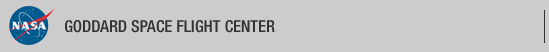+ Visit NASA.gov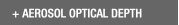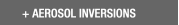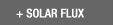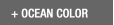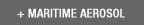Web Site Feature AERONET Data Synergy Tool - Access Earth Science data sets for AERONET sites+Home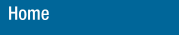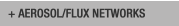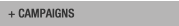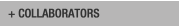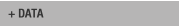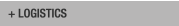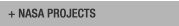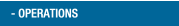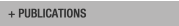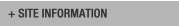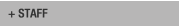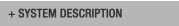AERONET DATA ACCESS

AERONET Site Lists (V3)

+ All Lists# Report of DCP transmissions

 Status Legend

AERONET No 6 [1317E33A] - 28 msg rcvd (4104 b) site : GSFC (Last Tx is before schedule by 0 min 3 sec
28 messages stepped outside their assigned time window)
AERONET No 26 [1316E1C0] - 28 msg rcvd (3777 b) site : GSFC (Last Tx is late by 0 min 1 sec)
AERONET No 28 [13101F58] - 28 msg rcvd (10105 b) site : White_Sands_HELSTF (Last Tx is precisely on time)
AERONET No 59 [131A03AE] - 28 msg rcvd (3941 b) site : Sevilleta (Last Tx is precisely on time)
AERONET No 134  - 28 msg rcvd (5348 b) site : GSFC (Last Tx is before schedule by 0 min 3 sec
28 messages stepped outside their assigned time window)
AERONET No 163  - 28 msg rcvd (17275 b) site : UCSB (Last Tx is precisely on time)
AERONET No 196 [1319A458] - 28 msg rcvd (18352 b) site : Rio_Branco (Last Tx is precisely on time)
AERONET No 306 [131A40A4] - 28 msg rcvd (5670 b) site : Easton-MDE (Last Tx is precisely on time , 29b)
AERONET No 307 [1318152C] - 28 msg rcvd (10865 b) site : Ames (Last Tx is late by 0 min 1 sec , 5b)
AERONET No 317 [131982B4] - 28 msg rcvd (11310 b) site : Tudor_Hill (Last Tx is precisely on time)
AERONET No 319 [131820B6] - 28 msg rcvd (7985 b) site : Trelew (Last Tx is late by 0 min 1 sec 276s)
AERONET No 330 [131481D2] - 28 msg rcvd (3954 b) site : GSFC (Last Tx is late by 0 min 1 sec)
AERONET No 365 [131A53D2] - 28 msg rcvd (12199 b) site : Ji_Parana_SE (Last Tx is precisely on time , 22b)
AERONET No 374 [131713BE] - 28 msg rcvd (18155 b) site : TABLE_MOUNTAIN_CA (Last Tx is precisely on time)
AERONET No 409 [131AA356] - 26 msg rcvd (4775 b) site : Mingo (Last Tx is precisely on time)
AERONET No 601 [1319E752] - 28 msg rcvd (3136 b) site : CCNY ()
AERONET No 624 [131AE05C] - 28 msg rcvd (3108 b) site : CUIABA-MIRANDA ()
AERONET No 635 [1319D2C8] - 28 msg rcvd (26280 b) site : Alta_Floresta (Last Tx is precisely on time)
AERONET No 707 [1316D45A] - 20 msg rcvd (1940 b) site : Ascension_Island (Last Tx is precisely on time)
AERONET No 813 [1310118A] - 16 msg rcvd (2877 b) site : Yuma (Last Tx is precisely on time)

# Aeronet No 6 [1317E33A] (GSFC)

Last Tx is before schedule by 0 min 3 sec
28 messages stepped outside their assigned time window

09:12:2019,10:41:47 (UTC) 'G' (DCP total: 87) Cimel : 6 time corr. = -24 sec (DCP Volt = 13.00 Lat= 38.993 Long= -76.840(0.1 km from GSFC))
09:12:2019,11:41:47 (UTC) 'G' (DCP total: 87) Cimel : 6 time corr. = -23 sec (DCP Volt = 13.00 Lat= 38.993 Long= -76.840(0.1 km from GSFC))
09:12:2019,12:41:47 (UTC) 'G' (DCP total: 87) Cimel : 6 time corr. = -23 sec (DCP Volt = 13.00 Lat= 38.993 Long= -76.840(0.0 km from GSFC))
09:12:2019,13:41:47 (UTC) 'G' (DCP total: 234) Cimel : 6 time corr. = -24 sec (DCP Volt = 13.00 Lat= 38.993 Long= -76.840(0.0 km from GSFC)) stah stah stah stah stah stah
09:12:2019,14:41:47 (UTC) 'G' (DCP total: 339) Cimel : 6 time corr. = -23 sec (DCP Volt = 13.00 Lat= 38.993 Long= -76.841(0.1 km from GSFC)) stah stah stah stah stah stah stah stah stah stah stah stah
09:12:2019,15:41:47 (UTC) 'G' (DCP total: 339) Cimel : 6 time corr. = -24 sec (DCP Volt = 13.00 Lat= 38.993 Long= -76.841(0.1 km from GSFC)) stah stah stah stah stah stah stah stah stah stah stah stah
09:12:2019,16:41:47 (UTC) 'G' (DCP total: 339) Cimel : 6 time corr. = -25 sec (DCP Volt = 13.00 Lat= 38.993 Long= -76.841(0.1 km from GSFC)) stah stah stah stah stah stah stah stah stah stah stah stah
09:12:2019,17:41:47 (UTC) 'G' (DCP total: 339) Cimel : 6 time corr. = -25 sec (DCP Volt = 13.00 Lat= 38.993 Long= -76.841(0.1 km from GSFC)) stah stah stah stah stah stah stah stah stah stah stah stah
09:12:2019,18:41:47 (UTC) 'G' (DCP total: 318) Cimel : 6 time corr. = -24 sec (DCP Volt = 13.00 Lat= 38.993 Long= -76.841(0.1 km from GSFC)) stah stah stah stah stah stah stah stah stah stah stah
09:12:2019,19:41:47 (UTC) 'G' (DCP total: 318) Cimel : 6 time corr. = -25 sec (DCP Volt = 13.00 Lat= 38.993 Long= -76.841(0.1 km from GSFC)) stah stah stah stah stah stah stah stah stah stah stah
09:12:2019,20:41:47 (UTC) 'G' (DCP total: 339) Cimel : 6 time corr. = -25 sec (DCP Volt = 13.00 Lat= 38.992 Long= -76.840(0.0 km from GSFC)) stah stah stah stah stah stah stah stah stah stah stah stah
09:12:2019,21:41:47 (UTC) 'G' (DCP total: 108) Cimel : 6 time corr. = -24 sec (DCP Volt = 13.00 Lat= 38.992 Long= -76.840(0.0 km from GSFC)) stah
09:12:2019,22:41:47 (UTC) 'G' (DCP total: 87) Cimel : 6 time corr. = -25 sec (DCP Volt = 13.00 Lat= 38.993 Long= -76.840(0.1 km from GSFC))
09:12:2019,23:41:47 (UTC) 'G' (DCP total: 87) Cimel : 6 time corr. = -25 sec (DCP Volt = 13.00 Lat= 38.993 Long= -76.840(0.1 km from GSFC))
10:12:2019,00:41:47 (UTC) 'G' (DCP total: 87) Cimel : 6 time corr. = -24 sec (DCP Volt = 13.00 Lat= 38.993 Long= -76.840(0.0 km from GSFC))
10:12:2019,01:41:47 (UTC) 'G' (DCP total: 87) Cimel : 6 time corr. = -25 sec (DCP Volt = 13.00 Lat= 38.993 Long= -76.840(0.0 km from GSFC))
10:12:2019,02:41:47 (UTC) 'G' (DCP total: 87) Cimel : 6 time corr. = -25 sec (DCP Volt = 13.00 Lat= 38.993 Long= -76.840(0.0 km from GSFC))
10:12:2019,03:41:47 (UTC) 'G' (DCP total: 87) Cimel : 6 time corr. = -24 sec (DCP Volt = 13.00 Lat= 38.992 Long= -76.840(0.0 km from GSFC))
10:12:2019,04:41:47 (UTC) 'G' (DCP total: 87) Cimel : 6 time corr. = -25 sec (DCP Volt = 13.00 Lat= 38.992 Long= -76.840(0.0 km from GSFC))
10:12:2019,05:41:47 (UTC) 'G' (DCP total: 87) Cimel : 6 time corr. = -25 sec (DCP Volt = 13.00 Lat= 38.992 Long= -76.840(0.0 km from GSFC))
10:12:2019,06:41:47 (UTC) 'G' (DCP total: 87) Cimel : 6 time corr. = -24 sec (DCP Volt = 13.00 Lat= 38.992 Long= -76.840(0.1 km from GSFC))
10:12:2019,07:41:47 (UTC) 'G' (DCP total: 87) Cimel : 6 time corr. = -26 sec (DCP Volt = 13.00 Lat= 38.993 Long= -76.840(0.0 km from GSFC))
10:12:2019,08:41:47 (UTC) 'G' (DCP total: 87) Cimel : 6 time corr. = -25 sec (DCP Volt = 13.00 Lat= 38.992 Long= -76.840(0.0 km from GSFC))
10:12:2019,09:41:47 (UTC) 'G' (DCP total: 87) Cimel : 6 time corr. = -25 sec (DCP Volt = 13.00 Lat= 38.993 Long= -76.840(0.0 km from GSFC))
10:12:2019,10:41:47 (UTC) 'G' (DCP total: 87) Cimel : 6 time corr. = -26 sec (DCP Volt = 13.00 Lat= 38.993 Long= -76.840(0.1 km from GSFC))
10:12:2019,11:41:47 (UTC) 'G' (DCP total: 87) Cimel : 6 time corr. = -25 sec (DCP Volt = 13.00 Lat= 38.993 Long= -76.840(0.1 km from GSFC))
10:12:2019,12:41:47 (UTC) 'G' (DCP total: 87) Cimel : 6 time corr. = -25 sec (DCP Volt = 13.00 Lat= 38.993 Long= -76.841(0.1 km from GSFC))
10:12:2019,13:41:47 (UTC) 'G' (DCP total: 313) Cimel : 6 time corr. = -26 sec (DCP Volt = 13.00 Lat= 38.993 Long= -76.840(0.0 km from GSFC)) stah staD nsu staD

# Aeronet No 26 [1316E1C0] (GSFC)

Last Tx is late by 0 min 1 sec

09:12:2019,10:32:41 (UTC) 'G' (DCP total: 89) Cimel : 26 time corr. = -1 sec (DCP Volt = 12.80 Lat= 38.993 Long= -76.840(0.0 km from GSFC))
09:12:2019,11:32:41 (UTC) 'G' (DCP total: 89) Cimel : 26 time corr. = -2 sec (DCP Volt = 12.80 Lat= 38.992 Long= -76.840(0.0 km from GSFC))
09:12:2019,12:32:41 (UTC) 'G' (DCP total: 89) Cimel : 26 time corr. = -1 sec (DCP Volt = 12.80 Lat= 38.993 Long= -76.840(0.0 km from GSFC))
09:12:2019,13:32:41 (UTC) 'G' (DCP total: 173) Cimel : 26 time corr. = -1 sec (DCP Volt = 12.80 Lat= 38.993 Long= -76.840(0.0 km from GSFC)) stah stah stah
09:12:2019,14:32:41 (UTC) 'G' (DCP total: 496) Cimel : 26 time corr. = -2 sec (DCP Volt = 12.80 Lat= 38.993 Long= -76.840(0.0 km from GSFC)) stah stah stah stah staA ssk staF nsu staD nsu
09:12:2019,15:32:41 (UTC) 'G' (DCP total: 325) Cimel : 26 time corr. = -1 sec (DCP Volt = 12.90 Lat= 38.993 Long= -76.840(0.0 km from GSFC)) staD nsu staD nsu stah stah
09:12:2019,16:32:41 (UTC) 'G' (DCP total: 173) Cimel : 26 time corr. = -1 sec (DCP Volt = 12.90 Lat= 38.993 Long= -76.840(0.0 km from GSFC)) stah stah stah stah
09:12:2019,17:32:41 (UTC) 'G' (DCP total: 173) Cimel : 26 time corr. = -2 sec (DCP Volt = 13.00 Lat= 38.992 Long= -76.840(0.0 km from GSFC)) stah stah stah stah
09:12:2019,18:32:41 (UTC) 'G' (DCP total: 173) Cimel : 26 time corr. = -1 sec (DCP Volt = 13.00 Lat= 38.993 Long= -76.840(0.0 km from GSFC)) stah stah stah stah
09:12:2019,19:32:41 (UTC) 'G' (DCP total: 173) Cimel : 26 time corr. = -1 sec (DCP Volt = 12.80 Lat= 38.993 Long= -76.840(0.0 km from GSFC)) stah stah stah stah
09:12:2019,20:32:41 (UTC) 'G' (DCP total: 215) Cimel : 26 time corr. = -2 sec (DCP Volt = 12.80 Lat= 38.992 Long= -76.840(0.0 km from GSFC)) stah stah stah stah stah stah
09:12:2019,21:32:41 (UTC) 'G' (DCP total: 173) Cimel : 26 time corr. = -1 sec (DCP Volt = 12.80 Lat= 38.992 Long= -76.840(0.0 km from GSFC)) stah stah stah stah
09:12:2019,22:32:41 (UTC) 'G' (DCP total: 89) Cimel : 26 time corr. = -1 sec (DCP Volt = 12.80 Lat= 38.993 Long= -76.840(0.0 km from GSFC))
09:12:2019,23:32:41 (UTC) 'G' (DCP total: 89) Cimel : 26 time corr. = -2 sec (DCP Volt = 12.80 Lat= 38.993 Long= -76.840(0.0 km from GSFC))
10:12:2019,00:32:41 (UTC) 'G' (DCP total: 89) Cimel : 26 time corr. = -1 sec (DCP Volt = 12.80 Lat= 38.993 Long= -76.840(0.0 km from GSFC))
10:12:2019,01:32:41 (UTC) 'G' (DCP total: 89) Cimel : 26 time corr. = -1 sec (DCP Volt = 12.80 Lat= 38.993 Long= -76.840(0.0 km from GSFC))
10:12:2019,02:32:41 (UTC) 'G' (DCP total: 89) Cimel : 26 time corr. = -2 sec (DCP Volt = 12.80 Lat= 38.993 Long= -76.840(0.0 km from GSFC))
10:12:2019,03:32:41 (UTC) 'G' (DCP total: 89) Cimel : 26 time corr. = -1 sec (DCP Volt = 12.80 Lat= 38.993 Long= -76.840(0.0 km from GSFC))
10:12:2019,04:32:41 (UTC) 'G' (DCP total: 89) Cimel : 26 time corr. = -1 sec (DCP Volt = 12.80 Lat= 38.993 Long= -76.840(0.0 km from GSFC))
10:12:2019,05:32:41 (UTC) 'G' (DCP total: 89) Cimel : 26 time corr. = -2 sec (DCP Volt = 12.80 Lat= 38.993 Long= -76.840(0.0 km from GSFC))
10:12:2019,06:32:41 (UTC) 'G' (DCP total: 89) Cimel : 26 time corr. = -1 sec (DCP Volt = 12.80 Lat= 38.993 Long= -76.840(0.0 km from GSFC))
10:12:2019,07:32:41 (UTC) 'G' (DCP total: 89) Cimel : 26 time corr. = -1 sec (DCP Volt = 12.80 Lat= 38.993 Long= -76.840(0.0 km from GSFC))
10:12:2019,08:32:41 (UTC) 'G' (DCP total: 89) Cimel : 26 time corr. = -2 sec (DCP Volt = 12.80 Lat= 38.993 Long= -76.840(0.0 km from GSFC))
10:12:2019,09:32:41 (UTC) 'G' (DCP total: 89) Cimel : 26 time corr. = -1 sec (DCP Volt = 12.80 Lat= 38.993 Long= -76.840(0.0 km from GSFC))
10:12:2019,10:32:41 (UTC) 'G' (DCP total: 89) Cimel : 26 time corr. = -1 sec (DCP Volt = 12.80 Lat= 38.993 Long= -76.840(0.0 km from GSFC))
10:12:2019,11:32:41 (UTC) 'G' (DCP total: 89) Cimel : 26 time corr. = -2 sec (DCP Volt = 12.80 Lat= 38.993 Long= -76.840(0.0 km from GSFC))
10:12:2019,12:32:41 (UTC) 'G' (DCP total: 89) Cimel : 26 time corr. = -1 sec (DCP Volt = 12.80 Lat= 38.993 Long= -76.840(0.0 km from GSFC))
10:12:2019,13:32:41 (UTC) 'G' (DCP total: 605) Cimel : 26 time corr. = -1 sec (DCP Volt = 12.80 Lat= 38.993 Long= -76.840(0.0 km from GSFC)) stah staA ssk staA ssk staA ssk staA

# Aeronet No 28 [13101F58] (White_Sands_HELSTF)

Last Tx is precisely on time

09:12:2019,10:12:21 (UTC) 'G' (DCP total: 88) Cimel : 28 time corr. = 2 sec (DCP Volt = 12.40 Lat= 32.635 Long= -106.339(0.1 km from White_Sands_HELSTF))
09:12:2019,11:12:21 (UTC) 'G' (DCP total: 88) Cimel : 28 time corr. = 2 sec (DCP Volt = 12.40 Lat= 32.635 Long= -106.339(0.1 km from White_Sands_HELSTF))
09:12:2019,12:12:21 (UTC) 'G' (DCP total: 88) Cimel : 28 time corr. = 0 sec (DCP Volt = 12.40 Lat= 32.635 Long= -106.339(0.1 km from White_Sands_HELSTF))
09:12:2019,13:12:21 (UTC) 'G' (DCP total: 88) Cimel : 28 time corr. = 1 sec (DCP Volt = 12.40 Lat= 32.635 Long= -106.339(0.1 km from White_Sands_HELSTF))
09:12:2019,14:12:21 (UTC) 'G' (DCP total: 88) Cimel : 28 time corr. = 0 sec (DCP Volt = 12.40 Lat= 32.635 Long= -106.339(0.1 km from White_Sands_HELSTF))
09:12:2019,15:12:21 (UTC) 'G' (DCP total: 573) Cimel : 28 time corr. = 0 sec (DCP Volt = 12.40 Lat= 32.635 Long= -106.339(0.1 km from White_Sands_HELSTF)) staA nsu staA nsu staA nsu staA nsu staA
09:12:2019,16:12:21 (UTC) 'G' (DCP total: 864) Cimel : 28 time corr. = 1 sec (DCP Volt = 12.40 Lat= 32.635 Long= -106.339(0.1 km from White_Sands_HELSTF)) nsu staA nsu staB nsu staA nsu staA nsu staA nsu staA nsu staC nsu staF
09:12:2019,17:12:21 (UTC) 'G' (DCP total: 476) Cimel : 28 time corr. = 0 sec (DCP Volt = 12.40 Lat= 32.635 Long= -106.339(0.1 km from White_Sands_HELSTF)) nsu staD nsu staD nsu staD nsu staF
09:12:2019,18:12:21 (UTC) 'G' (DCP total: 476) Cimel : 28 time corr. = 0 sec (DCP Volt = 12.40 Lat= 32.635 Long= -106.339(0.1 km from White_Sands_HELSTF)) nsu staD nsu staD nsu staD nsu staF
09:12:2019,19:12:21 (UTC) 'G' (DCP total: 476) Cimel : 28 time corr. = 1 sec (DCP Volt = 12.40 Lat= 32.635 Long= -106.339(0.1 km from White_Sands_HELSTF)) nsu staD nsu staD nsu staD nsu staF
09:12:2019,20:12:21 (UTC) 'G' (DCP total: 476) Cimel : 28 time corr. = 0 sec (DCP Volt = 12.40 Lat= 32.635 Long= -106.339(0.1 km from White_Sands_HELSTF)) nsu staD nsu staD nsu staD nsu staF
09:12:2019,21:12:21 (UTC) 'G' (DCP total: 476) Cimel : 28 time corr. = 0 sec (DCP Volt = 12.30 Lat= 32.635 Long= -106.339(0.1 km from White_Sands_HELSTF)) nsu staD nsu staD nsu staD nsu staF
09:12:2019,22:12:21 (UTC) 'G' (DCP total: 1212) Cimel : 28 time corr. = 1 sec (DCP Volt = 13.20 Lat= 32.635 Long= -106.339(0.1 km from White_Sands_HELSTF)) nsu staD nsu staD nsu staD nsu staF nsu all(1) alr(1) all(2) alr(2) all(3) alr(3) all(4)
09:12:2019,23:12:21 (UTC) 'G' (DCP total: 1214) Cimel : 28 time corr. = 0 sec (DCP Volt = 13.00 Lat= 32.635 Long= -106.339(0.1 km from White_Sands_HELSTF)) alr(4) nsu pp1(1) pp1(2) pp1(3) pp1(4) blk staC nsu all(1) alr(1) all(2) alr(2)
10:12:2019,00:12:21 (UTC) 'G' (DCP total: 1214) Cimel : 28 time corr. = 0 sec (DCP Volt = 12.70 Lat= 32.635 Long= -106.339(0.1 km from White_Sands_HELSTF)) all(3) alr(3) all(4) alr(4) nsu pp1(1) pp1(2) pp1(3) pp1(4) staA nsu staA nsu staA
10:12:2019,01:12:21 (UTC) 'G' (DCP total: 1212) Cimel : 28 time corr. = 1 sec (DCP Volt = 12.60 Lat= 32.635 Long= -106.339(0.1 km from White_Sands_HELSTF)) nsu staA nsu staB nsu all(1) alr(1) all(2) alr(2) all(3) alr(3) all(4) alr(4) staA nsu staA
10:12:2019,02:12:21 (UTC) 'G' (DCP total: 504) Cimel : 28 time corr. = 0 sec (DCP Volt = 12.50 Lat= 32.635 Long= -106.339(0.1 km from White_Sands_HELSTF)) nsu staA nsu staA nsu staA nsu staA
10:12:2019,03:12:21 (UTC) 'G' (DCP total: 88) Cimel : 28 time corr. = 0 sec (DCP Volt = 12.50 Lat= 32.635 Long= -106.339(0.1 km from White_Sands_HELSTF))
10:12:2019,04:12:21 (UTC) 'G' (DCP total: 88) Cimel : 28 time corr. = 1 sec (DCP Volt = 12.40 Lat= 32.635 Long= -106.339(0.1 km from White_Sands_HELSTF))
10:12:2019,05:12:21 (UTC) 'G' (DCP total: 88) Cimel : 28 time corr. = 0 sec (DCP Volt = 12.40 Lat= 32.635 Long= -106.339(0.1 km from White_Sands_HELSTF))
10:12:2019,06:12:21 (UTC) 'G' (DCP total: 88) Cimel : 28 time corr. = 0 sec (DCP Volt = 12.30 Lat= 32.635 Long= -106.339(0.1 km from White_Sands_HELSTF))
10:12:2019,07:12:21 (UTC) 'G' (DCP total: 88) Cimel : 28 time corr. = 1 sec (DCP Volt = 12.30 Lat= 32.635 Long= -106.339(0.1 km from White_Sands_HELSTF))
10:12:2019,08:12:21 (UTC) 'G' (DCP total: 88) Cimel : 28 time corr. = 0 sec (DCP Volt = 12.20 Lat= 32.635 Long= -106.339(0.1 km from White_Sands_HELSTF))
10:12:2019,09:12:21 (UTC) 'G' (DCP total: 88) Cimel : 28 time corr. = 0 sec (DCP Volt = 12.20 Lat= 32.635 Long= -106.339(0.1 km from White_Sands_HELSTF))
10:12:2019,10:12:21 (UTC) 'G' (DCP total: 88) Cimel : 28 time corr. = 1 sec (DCP Volt = 12.20 Lat= 32.635 Long= -106.339(0.1 km from White_Sands_HELSTF))
10:12:2019,11:12:21 (UTC) 'G' (DCP total: 88) Cimel : 28 time corr. = 0 sec (DCP Volt = 12.20 Lat= 32.635 Long= -106.339(0.1 km from White_Sands_HELSTF))
10:12:2019,12:12:21 (UTC) 'G' (DCP total: 88) Cimel : 28 time corr. = 0 sec (DCP Volt = 12.20 Lat= 32.635 Long= -106.339(0.1 km from White_Sands_HELSTF))
10:12:2019,13:12:21 (UTC) 'G' (DCP total: 88) Cimel : 28 time corr. = 1 sec (DCP Volt = 12.20 Lat= 32.635 Long= -106.339(0.1 km from White_Sands_HELSTF))

# Aeronet No 59 [131A03AE] (Sevilleta)

Last Tx is precisely on time

09:12:2019,10:23:01 (UTC) 'G' (DCP total: 89) Cimel : 59 time corr. = -3562 sec (DCP Volt = 12.70 Lat= 34.356 Long= -106.885(0.1 km from Sevilleta))
09:12:2019,11:23:01 (UTC) 'G' (DCP total: 89) Cimel : 59 time corr. = -3562 sec (DCP Volt = 12.70 Lat= 34.356 Long= -106.885(0.1 km from Sevilleta))
09:12:2019,12:23:01 (UTC) 'G' (DCP total: 89) Cimel : 59 time corr. = -3562 sec (DCP Volt = 12.70 Lat= 34.356 Long= -106.885(0.1 km from Sevilleta))
09:12:2019,13:23:01 (UTC) 'G' (DCP total: 89) Cimel : 59 time corr. = -3562 sec (DCP Volt = 12.70 Lat= 34.356 Long= -106.885(0.1 km from Sevilleta))
09:12:2019,14:23:01 (UTC) 'G' (DCP total: 278) Cimel : 59 time corr. = -3562 sec (DCP Volt = 12.70 Lat= 34.356 Long= -106.885(0.1 km from Sevilleta)) sta@ sta@ sta@ sta@ sta@ sta@ sta@ sta@ Date is wrong 6916 days in past
09:12:2019,15:23:01 (UTC) 'G' (DCP total: 425) Cimel : 59 time corr. = -3562 sec (DCP Volt = 12.60 Lat= 34.356 Long= -106.885(0.1 km from Sevilleta)) sta@ sta@ sta@ sta@ sta@ sta@ sta@ sta@ sta@ sta@ sta@ sta@ sta@ sta@ sta@ sta@ Date is wrong 6916 days in past
09:12:2019,16:23:01 (UTC) 'G' (DCP total: 257) Cimel : 59 time corr. = -3562 sec (DCP Volt = 12.70 Lat= 34.356 Long= -106.885(0.1 km from Sevilleta)) sta@ sta@ sta@ sta@ sta@ sta@ sta@ sta@ Date is wrong 6916 days in past
09:12:2019,17:23:01 (UTC) 'G' (DCP total: 257) Cimel : 59 time corr. = -3562 sec (DCP Volt = 13.20 Lat= 34.356 Long= -106.885(0.1 km from Sevilleta)) sta@ sta@ sta@ sta@ sta@ sta@ sta@ sta@ Date is wrong 6916 days in past
09:12:2019,18:23:01 (UTC) 'G' (DCP total: 257) Cimel : 59 time corr. = -3562 sec (DCP Volt = 13.50 Lat= 34.356 Long= -106.885(0.1 km from Sevilleta)) sta@ sta@ sta@ sta@ sta@ sta@ sta@ sta@ Date is wrong 6916 days in past
09:12:2019,19:23:01 (UTC) 'G' (DCP total: 257) Cimel : 59 time corr. = -3562 sec (DCP Volt = 14.00 Lat= 34.356 Long= -106.885(0.1 km from Sevilleta)) sta@ sta@ sta@ sta@ sta@ sta@ sta@ sta@ Date is wrong 6916 days in past
09:12:2019,20:23:01 (UTC) 'G' (DCP total: 257) Cimel : 59 time corr. = -3562 sec (DCP Volt = 14.10 Lat= 34.356 Long= -106.885(0.1 km from Sevilleta)) sta@ sta@ sta@ sta@ sta@ sta@ sta@ sta@ Date is wrong 6916 days in past
09:12:2019,21:23:01 (UTC) 'G' (DCP total: 341) Cimel : 59 time corr. = -3562 sec (DCP Volt = 14.10 Lat= 34.356 Long= -106.885(0.1 km from Sevilleta)) sta@ sta@ sta@ sta@ sta@ sta@ sta@ sta@ sta@ sta@ sta@ sta@ Date is wrong 6916 days in past
09:12:2019,22:23:01 (UTC) 'G' (DCP total: 425) Cimel : 59 time corr. = -3562 sec (DCP Volt = 13.90 Lat= 34.356 Long= -106.885(0.1 km from Sevilleta)) sta@ sta@ sta@ sta@ sta@ sta@ sta@ sta@ sta@ sta@ sta@ sta@ sta@ sta@ sta@ sta@ Date is wrong 6916 days in past
09:12:2019,23:23:01 (UTC) 'G' (DCP total: 89) Cimel : 59 time corr. = -3562 sec (DCP Volt = 14.10 Lat= 34.356 Long= -106.885(0.1 km from Sevilleta))
10:12:2019,00:23:01 (UTC) 'G' (DCP total: 89) Cimel : 59 time corr. = -3562 sec (DCP Volt = 13.70 Lat= 34.356 Long= -106.885(0.1 km from Sevilleta))
10:12:2019,01:23:01 (UTC) 'G' (DCP total: 89) Cimel : 59 time corr. = -3562 sec (DCP Volt = 13.00 Lat= 34.356 Long= -106.885(0.1 km from Sevilleta))
10:12:2019,02:23:01 (UTC) 'G' (DCP total: 89) Cimel : 59 time corr. = -3562 sec (DCP Volt = 12.80 Lat= 34.356 Long= -106.885(0.1 km from Sevilleta))
10:12:2019,03:23:01 (UTC) 'G' (DCP total: 89) Cimel : 59 time corr. = -3562 sec (DCP Volt = 12.80 Lat= 34.356 Long= -106.885(0.1 km from Sevilleta))
10:12:2019,04:23:01 (UTC) 'G' (DCP total: 89) Cimel : 59 time corr. = -3562 sec (DCP Volt = 12.80 Lat= 34.356 Long= -106.885(0.1 km from Sevilleta))
10:12:2019,05:23:01 (UTC) 'G' (DCP total: 89) Cimel : 59 time corr. = -3562 sec (DCP Volt = 12.80 Lat= 34.356 Long= -106.885(0.1 km from Sevilleta))
10:12:2019,06:23:01 (UTC) 'G' (DCP total: 89) Cimel : 59 time corr. = -3562 sec (DCP Volt = 12.80 Lat= 34.356 Long= -106.885(0.1 km from Sevilleta))
10:12:2019,07:23:01 (UTC) 'G' (DCP total: 89) Cimel : 59 time corr. = -3562 sec (DCP Volt = 12.70 Lat= 34.356 Long= -106.885(0.1 km from Sevilleta))
10:12:2019,08:23:01 (UTC) 'G' (DCP total: 89) Cimel : 59 time corr. = -3562 sec (DCP Volt = 12.70 Lat= 34.356 Long= -106.885(0.1 km from Sevilleta))
10:12:2019,09:23:01 (UTC) 'G' (DCP total: 89) Cimel : 59 time corr. = -3562 sec (DCP Volt = 12.70 Lat= 34.356 Long= -106.885(0.1 km from Sevilleta))
10:12:2019,10:23:01 (UTC) 'G' (DCP total: 89) Cimel : 59 time corr. = -3562 sec (DCP Volt = 12.70 Lat= 34.356 Long= -106.885(0.1 km from Sevilleta))
10:12:2019,11:23:01 (UTC) 'G' (DCP total: 89) Cimel : 59 time corr. = -3562 sec (DCP Volt = 12.70 Lat= 34.356 Long= -106.885(0.1 km from Sevilleta))
10:12:2019,12:23:01 (UTC) 'G' (DCP total: 89) Cimel : 59 time corr. = -3562 sec (DCP Volt = 12.60 Lat= 34.356 Long= -106.885(0.1 km from Sevilleta))
10:12:2019,13:23:01 (UTC) 'G' (DCP total: 89) Cimel : 59 time corr. = -3562 sec (DCP Volt = 12.60 Lat= 34.356 Long= -106.885(0.1 km from Sevilleta))

# Aeronet No 134  (GSFC)

Last Tx is before schedule by 0 min 3 sec
28 messages stepped outside their assigned time window

09:12:2019,10:30:38 (UTC) 'G' (DCP total: 88) Cimel : 134 time corr. = 9 sec (DCP Volt = 11.80 Lat= 38.993 Long= -76.840(0.1 km from GSFC))
09:12:2019,11:30:38 (UTC) 'G' (DCP total: 88) Cimel : 134 time corr. = 9 sec (DCP Volt = 11.80 Lat= 38.992 Long= -76.840(0.0 km from GSFC))
09:12:2019,12:30:38 (UTC) 'G' (DCP total: 88) Cimel : 134 time corr. = 9 sec (DCP Volt = 11.80 Lat= 38.993 Long= -76.840(0.0 km from GSFC))
09:12:2019,13:30:38 (UTC) 'G' (DCP total: 604) Cimel : 134 time corr. = 9 sec (DCP Volt = 11.80 Lat= 38.992 Long= -76.840(0.0 km from GSFC)) staA ssk staA ssk staA ssk staA
09:12:2019,14:30:38 (UTC) 'G' (DCP total: 819) Cimel : 134 time corr. = 9 sec (DCP Volt = 11.80 Lat= 38.992 Long= -76.840(0.0 km from GSFC)) ssk staA ssk staA ssk staB ssk staA ssk staF nsu staD nsu
09:12:2019,15:30:38 (UTC) 'G' (DCP total: 455) Cimel : 134 time corr. = 9 sec (DCP Volt = 12.00 Lat= 38.993 Long= -76.840(0.0 km from GSFC)) staD nsu staD nsu staF nsu staD
09:12:2019,16:30:38 (UTC) 'G' (DCP total: 476) Cimel : 134 time corr. = 9 sec (DCP Volt = 12.00 Lat= 38.993 Long= -76.840(0.0 km from GSFC)) nsu staD nsu staD nsu staF nsu staD
09:12:2019,17:30:38 (UTC) 'G' (DCP total: 476) Cimel : 134 time corr. = 9 sec (DCP Volt = 12.00 Lat= 38.993 Long= -76.840(0.1 km from GSFC)) nsu staD nsu staD nsu staF nsu staD
09:12:2019,18:30:38 (UTC) 'G' (DCP total: 476) Cimel : 134 time corr. = 9 sec (DCP Volt = 12.10 Lat= 38.992 Long= -76.840(0.0 km from GSFC)) nsu staD nsu staD nsu staF nsu staD
09:12:2019,19:30:38 (UTC) 'G' (DCP total: 476) Cimel : 134 time corr. = 9 sec (DCP Volt = 12.00 Lat= 38.993 Long= -76.839(0.1 km from GSFC)) nsu staD nsu staD nsu staF nsu staD
09:12:2019,20:30:38 (UTC) 'G' (DCP total: 282) Cimel : 134 time corr. = 9 sec (DCP Volt = 12.00 Lat= 38.993 Long= -76.840(0.0 km from GSFC)) nsu staD nsu staD
09:12:2019,21:30:38 (UTC) 'G' (DCP total: 88) Cimel : 134 time corr. = 9 sec (DCP Volt = 11.80 Lat= 38.993 Long= -76.840(0.0 km from GSFC))
09:12:2019,22:30:38 (UTC) 'G' (DCP total: 88) Cimel : 134 time corr. = 9 sec (DCP Volt = 11.80 Lat= 38.993 Long= -76.840(0.0 km from GSFC))
09:12:2019,23:30:38 (UTC) 'G' (DCP total: 88) Cimel : 134 time corr. = 9 sec (DCP Volt = 11.80 Lat= 38.993 Long= -76.840(0.0 km from GSFC))
10:12:2019,00:30:38 (UTC) 'G' (DCP total: 88) Cimel : 134 time corr. = 9 sec (DCP Volt = 11.80 Lat= 38.992 Long= -76.840(0.0 km from GSFC))
10:12:2019,01:30:38 (UTC) 'G' (DCP total: 88) Cimel : 134 time corr. = 9 sec (DCP Volt = 11.80 Lat= 38.992 Long= -76.840(0.0 km from GSFC))
10:12:2019,02:30:38 (UTC) 'G' (DCP total: 88) Cimel : 134 time corr. = 9 sec (DCP Volt = 11.80 Lat= 38.992 Long= -76.840(0.0 km from GSFC))
10:12:2019,03:30:38 (UTC) 'G' (DCP total: 88) Cimel : 134 time corr. = 9 sec (DCP Volt = 11.80 Lat= 38.992 Long= -76.840(0.0 km from GSFC))
10:12:2019,04:30:38 (UTC) 'G' (DCP total: 88) Cimel : 134 time corr. = 9 sec (DCP Volt = 11.80 Lat= 38.993 Long= -76.840(0.0 km from GSFC))
10:12:2019,05:30:38 (UTC) 'G' (DCP total: 88) Cimel : 134 time corr. = 9 sec (DCP Volt = 11.80 Lat= 38.993 Long= -76.840(0.0 km from GSFC))
10:12:2019,06:30:38 (UTC) 'G' (DCP total: 88) Cimel : 134 time corr. = 9 sec (DCP Volt = 11.80 Lat= 38.993 Long= -76.840(0.0 km from GSFC))
10:12:2019,07:30:38 (UTC) 'G' (DCP total: 88) Cimel : 134 time corr. = 9 sec (DCP Volt = 11.80 Lat= 38.993 Long= -76.840(0.0 km from GSFC))
10:12:2019,08:30:38 (UTC) 'G' (DCP total: 88) Cimel : 134 time corr. = 9 sec (DCP Volt = 11.80 Lat= 38.993 Long= -76.840(0.0 km from GSFC))
10:12:2019,09:30:38 (UTC) 'G' (DCP total: 88) Cimel : 134 time corr. = 9 sec (DCP Volt = 11.80 Lat= 38.993 Long= -76.840(0.0 km from GSFC))
10:12:2019,10:30:38 (UTC) 'G' (DCP total: 88) Cimel : 134 time corr. = 9 sec (DCP Volt = 11.80 Lat= 38.993 Long= -76.840(0.0 km from GSFC))
10:12:2019,11:30:38 (UTC) 'G' (DCP total: 88) Cimel : 134 time corr. = 9 sec (DCP Volt = 11.80 Lat= 38.993 Long= -76.840(0.0 km from GSFC))
10:12:2019,12:30:38 (UTC) 'G' (DCP total: 88) Cimel : 134 time corr. = 9 sec (DCP Volt = 11.70 Lat= 38.992 Long= -76.840(0.0 km from GSFC))
10:12:2019,13:30:38 (UTC) 'G' (DCP total: 88) Cimel : 134 time corr. = 9 sec (DCP Volt = 11.80 Lat= 38.992 Long= -76.840(0.0 km from GSFC))

# Aeronet No 163  (UCSB)

Last Tx is precisely on time

09:12:2019,10:39:51 (UTC) 'G' (DCP total: 90) Cimel : 163 time corr. = 22 sec (DCP Volt = 12.70 Lat= 34.415 Long= -119.845(0.0 km from UCSB))
09:12:2019,11:39:51 (UTC) 'G' (DCP total: 90) Cimel : 163 time corr. = 22 sec (DCP Volt = 12.70 Lat= 34.415 Long= -119.845(0.0 km from UCSB))
09:12:2019,12:39:51 (UTC) 'G' (DCP total: 90) Cimel : 163 time corr. = 22 sec (DCP Volt = 12.70 Lat= 34.415 Long= -119.845(0.0 km from UCSB))
09:12:2019,13:39:51 (UTC) 'G' (DCP total: 90) Cimel : 163 time corr. = 22 sec (DCP Volt = 12.60 Lat= 34.415 Long= -119.845(0.0 km from UCSB))
09:12:2019,14:39:51 (UTC) 'G' (DCP total: 90) Cimel : 163 time corr. = 22 sec (DCP Volt = 12.60 Lat= 34.415 Long= -119.845(0.0 km from UCSB))
09:12:2019,15:39:51 (UTC) 'G' (DCP total: 90) Cimel : 163 time corr. = 22 sec (DCP Volt = 12.60 Lat= 34.415 Long= -119.845(0.0 km from UCSB))
09:12:2019,16:39:51 (UTC) 'G' (DCP total: 1214) Cimel : 163 time corr. = 22 sec (DCP Volt = 12.50 Lat= 34.415 Long= -119.845(0.0 km from UCSB)) staA nsu staA nsu staA nsu staA nsu staA nsu staA nsu staB nsu all(1) alr(1) all(2) alr(2)
09:12:2019,17:39:51 (UTC) 'G' (DCP total: 1214) Cimel : 163 time corr. = 23 sec (DCP Volt = 13.40 Lat= 34.415 Long= -119.845(0.0 km from UCSB)) all(3) alr(3) all(4) alr(4) staA nsu staA nsu staA nsu staA nsu staF nsu all(1) alr(1) all(2)
09:12:2019,18:39:51 (UTC) 'G' (DCP total: 1216) Cimel : 163 time corr. = 22 sec (DCP Volt = 13.80 Lat= 34.415 Long= -119.845(0.0 km from UCSB)) alr(2) all(3) alr(3) all(4) alr(4) nsu pp1(1) pp1(2) pp1(3) pp1(4) blk staD nsu staD
09:12:2019,19:39:51 (UTC) 'G' (DCP total: 1217) Cimel : 163 time corr. = 22 sec (DCP Volt = 13.80 Lat= 34.415 Long= -119.845(0.0 km from UCSB)) nsu staD nsu staF nsu all(1) alr(1) all(2) alr(2) all(3) alr(3) all(4) alr(4) nsu
09:12:2019,20:39:51 (UTC) 'G' (DCP total: 1217) Cimel : 163 time corr. = 23 sec (DCP Volt = 13.80 Lat= 34.415 Long= -119.845(0.0 km from UCSB)) pp1(1) pp1(2) pp1(3) pp1(4) blk staD nsu staD nsu staD nsu staF nsu all(1) alr(1) all(2)
09:12:2019,21:39:51 (UTC) 'G' (DCP total: 1216) Cimel : 163 time corr. = 22 sec (DCP Volt = 13.60 Lat= 34.415 Long= -119.845(0.0 km from UCSB)) alr(2) all(3) alr(3) all(4) alr(4) nsu pp1(1) pp1(2) pp1(3) pp1(4) blk staD nsu
09:12:2019,22:39:51 (UTC) 'G' (DCP total: 1217) Cimel : 163 time corr. = 22 sec (DCP Volt = 13.40 Lat= 34.415 Long= -119.845(0.0 km from UCSB)) staD nsu staD nsu staF nsu all(1) alr(1) all(2) alr(2) all(3) alr(3) all(4) alr(4) nsu
09:12:2019,23:39:51 (UTC) 'G' (DCP total: 1216) Cimel : 163 time corr. = 23 sec (DCP Volt = 13.20 Lat= 34.415 Long= -119.845(0.0 km from UCSB)) pp1(1) pp1(2) pp1(3) pp1(4) blk staD nsu staD nsu staD nsu staF nsu all(1) alr(1)
10:12:2019,00:39:51 (UTC) 'G' (DCP total: 1216) Cimel : 163 time corr. = 22 sec (DCP Volt = 13.10 Lat= 34.415 Long= -119.845(0.0 km from UCSB)) all(2) alr(2) all(3) alr(3) all(4) alr(4) nsu pp1(1) pp1(2) pp1(3) pp1(4) blk staD
10:12:2019,01:39:51 (UTC) 'G' (DCP total: 1217) Cimel : 163 time corr. = 22 sec (DCP Volt = 12.80 Lat= 34.415 Long= -119.845(0.0 km from UCSB)) nsu staD nsu staD nsu staF nsu all(1) alr(1) all(2) alr(2) all(3) alr(3) all(4) alr(4)
10:12:2019,02:39:51 (UTC) 'G' (DCP total: 1217) Cimel : 163 time corr. = 23 sec (DCP Volt = 12.80 Lat= 34.415 Long= -119.845(0.0 km from UCSB)) nsu pp1(1) pp1(2) pp1(3) pp1(4) blk staD nsu staD nsu staD nsu staF nsu all(1) alr(1)
10:12:2019,03:39:51 (UTC) 'G' (DCP total: 1216) Cimel : 163 time corr. = 22 sec (DCP Volt = 12.80 Lat= 34.415 Long= -119.845(0.0 km from UCSB)) all(2) alr(2) all(3) alr(3) all(4) alr(4) nsu pp1(1) pp1(2) pp1(3) pp1(4) blk staA
10:12:2019,04:39:51 (UTC) 'G' (DCP total: 1214) Cimel : 163 time corr. = 22 sec (DCP Volt = 12.80 Lat= 34.415 Long= -119.845(0.0 km from UCSB)) nsu staA nsu staA nsu staA nsu staB nsu all(1) alr(1) all(2) alr(2) all(3) alr(3) all(4)
10:12:2019,05:39:51 (UTC) 'G' (DCP total: 740) Cimel : 163 time corr. = 23 sec (DCP Volt = 12.70 Lat= 34.415 Long= -119.845(0.0 km from UCSB)) alr(4) staA nsu staA nsu staA nsu staA nsu staA nsu staA
10:12:2019,06:39:51 (UTC) 'G' (DCP total: 90) Cimel : 163 time corr. = 22 sec (DCP Volt = 12.70 Lat= 34.415 Long= -119.845(0.0 km from UCSB))
10:12:2019,07:39:51 (UTC) 'G' (DCP total: 90) Cimel : 163 time corr. = 22 sec (DCP Volt = 12.70 Lat= 34.415 Long= -119.845(0.0 km from UCSB))
10:12:2019,08:39:51 (UTC) 'G' (DCP total: 90) Cimel : 163 time corr. = 23 sec (DCP Volt = 12.70 Lat= 34.415 Long= -119.845(0.0 km from UCSB))
10:12:2019,09:39:51 (UTC) 'G' (DCP total: 90) Cimel : 163 time corr. = 22 sec (DCP Volt = 12.70 Lat= 34.415 Long= -119.845(0.0 km from UCSB))
10:12:2019,10:39:51 (UTC) 'G' (DCP total: 90) Cimel : 163 time corr. = 24 sec (DCP Volt = 12.70 Lat= 34.415 Long= -119.845(0.0 km from UCSB))
10:12:2019,11:39:51 (UTC) 'G' (DCP total: 90) Cimel : 163 time corr. = 24 sec (DCP Volt = 12.70 Lat= 34.415 Long= -119.845(0.0 km from UCSB))
10:12:2019,12:39:51 (UTC) 'G' (DCP total: 90) Cimel : 163 time corr. = 23 sec (DCP Volt = 12.60 Lat= 34.415 Long= -119.845(0.0 km from UCSB))
10:12:2019,13:39:51 (UTC) 'G' (DCP total: 90) Cimel : 163 time corr. = 24 sec (DCP Volt = 12.60 Lat= 34.415 Long= -119.845(0.0 km from UCSB))

# Aeronet No 196 [1319A458] (Rio_Branco)

Last Tx is precisely on time

09:12:2019,10:22:01 (UTC) 'G' (DCP total: 188) tranms # : 197 Instrument number changed ID = P846 (1 sensor)
09:12:2019,11:22:01 (UTC) 'G' (DCP total: 179) tranms # : 197 Instrument number changed ID = P846 (1 sensor)
09:12:2019,12:22:01 (UTC) 'G' (DCP total: 211) tranms # : 197 Instrument number changed ID = P846 (1 sensor)
09:12:2019,13:22:01 (UTC) 'G' (DCP total: 218) tranms # : 197 Instrument number changed ID = P846 (1 sensor)
09:12:2019,14:22:01 (UTC) 'G' (DCP total: 219) tranms # : 197 corrected to Cimel : 196 time corr. was 33585 Processing delayed due to possible configuration change ID = P846 (1 sensor)
09:12:2019,15:22:01 (UTC) 'G' (DCP total: 219) Cimel : 196 time corr. = -20 sec ID = P846 (1 sensor) (DCP Volt = 13.70 Lat= -9.957 Long= -67.869(0.0 km from Rio_Branco))
09:12:2019,16:22:01 (UTC) 'G' (DCP total: 1193) Cimel : 196 time corr. = -19 sec ID = P846 (1 sensor) (DCP Volt = 13.70 Lat= -9.957 Long= -67.869(0.0 km from Rio_Branco)) staF nsu all(1) alr(1) all(2) alr(2) all(3) alr(3) all(4) alr(4) nsu
09:12:2019,17:22:01 (UTC) 'G' (DCP total: 1193) Cimel : 196 time corr. = -20 sec ID = P846 (1 sensor) (DCP Volt = 13.70 Lat= -9.957 Long= -67.869(0.0 km from Rio_Branco)) pp1(1) pp1(2) pp1(3) pp1(4) blk staD nsu staD nsu staD nsu staE nsu pp1(1)
09:12:2019,18:22:01 (UTC) 'G' (DCP total: 1195) Cimel : 196 time corr. = -20 sec ID = P846 (1 sensor) (DCP Volt = 13.70 Lat= -9.957 Long= -67.869(0.0 km from Rio_Branco)) pp1(2) pp1(3) pp1(4) blk staD nsu staD nsu staD nsu staF nsu all(1) alr(1)
09:12:2019,19:22:01 (UTC) 'G' (DCP total: 1193) Cimel : 196 time corr. = -19 sec ID = P846 (1 sensor) (DCP Volt = 13.70 Lat= -9.957 Long= -67.869(0.0 km from Rio_Branco)) all(2) alr(2) all(3) alr(3) all(4) alr(4) nsu pp1(1) pp1(2) pp1(3)
09:12:2019,20:22:01 (UTC) 'G' (DCP total: 1193) Cimel : 196 time corr. = -20 sec ID = P846 (1 sensor) (DCP Volt = 13.70 Lat= -9.957 Long= -67.869(0.0 km from Rio_Branco)) pp1(4) blk staD nsu staD nsu staD nsu staF nsu all(1) alr(1) all(2) alr(2)
09:12:2019,21:22:01 (UTC) 'G' (DCP total: 1194) Cimel : 196 time corr. = -20 sec ID = P846 (1 sensor) (DCP Volt = 13.70 Lat= -9.957 Long= -67.869(0.0 km from Rio_Branco)) all(3) alr(3) all(4) alr(4) nsu pp1(1) pp1(2) pp1(3) pp1(4) blk staD nsu
09:12:2019,22:22:01 (UTC) 'G' (DCP total: 1173) Cimel : 196 time corr. = -19 sec ID = P846 (1 sensor) (DCP Volt = 13.20 Lat= -9.957 Long= -67.869(0.0 km from Rio_Branco)) staD nsu staD nsu staE nsu pp1(1) pp1(2) pp1(3) pp1(4) blk staD nsu staD
09:12:2019,23:22:01 (UTC) 'G' (DCP total: 1159) Cimel : 196 time corr. = -20 sec ID = P846 (1 sensor) (DCP Volt = 13.60 Lat= -9.957 Long= -67.869(0.0 km from Rio_Branco)) nsu sky2 sky2 sky2 sky2 sky2 sky2 sky2 sky2 sky2 sky2 blk staB ssk all(1) alr(1) all(2) alr(2) all(3)
10:12:2019,00:22:01 (UTC) 'G' (DCP total: 1164) Cimel : 196 time corr. = -20 sec ID = P846 (1 sensor) (DCP Volt = 13.60 Lat= -9.957 Long= -67.869(0.0 km from Rio_Branco)) alr(3) all(4) alr(4) blk staB ssk all(1) alr(1) all(2) alr(2) all(3)
10:12:2019,01:22:01 (UTC) 'G' (DCP total: 1164) Cimel : 196 time corr. = -19 sec ID = P846 (1 sensor) (DCP Volt = 13.60 Lat= -9.957 Long= -67.869(0.0 km from Rio_Branco)) alr(3) all(4) alr(4) blk staA ssk staA ssk staA ssk staA ssk staC ssk all(1)
10:12:2019,02:22:01 (UTC) 'G' (DCP total: 1164) Cimel : 196 time corr. = -20 sec ID = P846 (1 sensor) (DCP Volt = 13.60 Lat= -9.957 Long= -67.869(0.0 km from Rio_Branco)) alr(1) all(2) alr(2) all(3) alr(3) all(4) alr(4) nsu pp1(1) pp1(2)
10:12:2019,03:22:01 (UTC) 'G' (DCP total: 1164) Cimel : 196 time corr. = -20 sec ID = P846 (1 sensor) (DCP Volt = 13.60 Lat= -9.957 Long= -67.869(0.0 km from Rio_Branco)) pp1(3) pp1(4) blk staA ssk staA ssk staA ssk staA ssk staB ssk
10:12:2019,04:22:01 (UTC) 'G' (DCP total: 1165) Cimel : 196 time corr. = -19 sec ID = P846 (1 sensor) (DCP Volt = 13.70 Lat= -9.957 Long= -67.869(0.0 km from Rio_Branco)) all(1) alr(1) all(2) alr(2) all(3) alr(3) all(4) alr(4) blk staA ssk staA ssk staA
10:12:2019,05:22:01 (UTC) 'G' (DCP total: 672) Cimel : 196 time corr. = -20 sec ID = P846 (1 sensor) (DCP Volt = 13.70 Lat= -9.957 Long= -67.869(0.0 km from Rio_Branco)) ssk staA ssk staA ssk staA
10:12:2019,06:22:01 (UTC) 'G' (DCP total: 189) Cimel : 196 time corr. = -20 sec ID = P846 (1 sensor) (DCP Volt = 13.70 Lat= -9.957 Long= -67.869(0.0 km from Rio_Branco))
10:12:2019,07:22:01 (UTC) 'G' (DCP total: 189) Cimel : 196 time corr. = -19 sec ID = P846 (1 sensor) (DCP Volt = 13.70 Lat= -9.957 Long= -67.869(0.0 km from Rio_Branco))
10:12:2019,08:22:01 (UTC) 'G' (DCP total: 189) Cimel : 196 time corr. = -20 sec ID = P846 (1 sensor) (DCP Volt = 13.70 Lat= -9.957 Long= -67.869(0.0 km from Rio_Branco))
10:12:2019,09:22:01 (UTC) 'G' (DCP total: 189) Cimel : 196 time corr. = -20 sec ID = P846 (1 sensor) (DCP Volt = 13.60 Lat= -9.957 Long= -67.869(0.0 km from Rio_Branco))
10:12:2019,10:22:01 (UTC) 'G' (DCP total: 184) Cimel : 196 time corr. = -19 sec ID = P846 (1 sensor) (DCP Volt = 13.70 Lat= -9.957 Long= -67.869(0.0 km from Rio_Branco))
10:12:2019,11:22:01 (UTC) 'G' (DCP total: 1172) Cimel : 196 time corr. = -20 sec ID = P846 (1 sensor) (DCP Volt = 13.70 Lat= -9.957 Long= -67.869(0.0 km from Rio_Branco)) ssk staA ssk sky2 sky2 sky2 sky2 sky2 sky2 sky2 sky2 staA ssk sky2 sky2 sky2 sky2 sky2 sky2 sky2 sky2 staA ssk sky2
10:12:2019,12:22:01 (UTC) 'G' (DCP total: 1193) Cimel : 196 time corr. = -20 sec ID = P846 (1 sensor) (DCP Volt = 13.30 Lat= -9.958 Long= -67.869(0.0 km from Rio_Branco)) sky2 sky2 sky2 sky2 sky2 sky2 sky2 sky2 sky2 blk staA ssk staA ssk staA ssk staB ssk all(1)
10:12:2019,13:22:01 (UTC) 'G' (DCP total: 1194) Cimel : 196 time corr. = -19 sec ID = P846 (1 sensor) (DCP Volt = 13.30 Lat= -9.957 Long= -67.869(0.0 km from Rio_Branco)) alr(1) all(2) alr(2) all(3) alr(3) all(4) alr(4) blk staA ssk staA ssk staA

# Aeronet No 306 [131A40A4] (Easton-MDE)

Last Tx is precisely on time

09:12:2019,10:23:41 (UTC) 'G' (DCP total: 87) Cimel : 306 time corr. = 44 sec (DCP Volt = 12.00 Lat= 38.795 Long= -76.084(0.0 km from Easton-MDE))
09:12:2019,11:23:41 (UTC) 'G' (DCP total: 87) Cimel : 306 time corr. = 44 sec (DCP Volt = 12.00 Lat= 38.795 Long= -76.084(0.0 km from Easton-MDE))
09:12:2019,12:23:41 (UTC) 'G' (DCP total: 87) Cimel : 306 time corr. = 44 sec (DCP Volt = 12.00 Lat= 38.795 Long= -76.084(0.0 km from Easton-MDE))
09:12:2019,13:23:41 (UTC) 'G' (DCP total: 386) Cimel : 306 time corr. = 44 sec (DCP Volt = 12.00 Lat= 38.795 Long= -76.084(0.0 km from Easton-MDE)) staA nsu stab staA nsu stab stah stab
09:12:2019,14:23:41 (UTC) 'G' (DCP total: 234) Cimel : 306 time corr. = 44 sec (DCP Volt = 12.00 Lat= 38.795 Long= -76.084(0.0 km from Easton-MDE)) stah stah stah stah stab stah stah
09:12:2019,15:23:41 (UTC) 'G' (DCP total: 171) Cimel : 306 time corr. = 44 sec (DCP Volt = 12.00 Lat= 38.795 Long= -76.084(0.0 km from Easton-MDE)) stah stah stah stah
09:12:2019,16:23:41 (UTC) 'G' (DCP total: 365) Cimel : 306 time corr. = 44 sec (DCP Volt = 12.00 Lat= 38.795 Long= -76.084(0.0 km from Easton-MDE)) stah stah stah staF nsu stab staD nsu
09:12:2019,17:23:41 (UTC) 'G' (DCP total: 365) Cimel : 306 time corr. = 44 sec (DCP Volt = 12.00 Lat= 38.795 Long= -76.084(0.0 km from Easton-MDE)) stab staD nsu stab stah stah staD nsu
09:12:2019,18:23:41 (UTC) 'G' (DCP total: 559) Cimel : 306 time corr. = 44 sec (DCP Volt = 12.00 Lat= 38.795 Long= -76.084(0.0 km from Easton-MDE)) stab staD nsu stab staD nsu stab staF nsu stab staD nsu
09:12:2019,19:23:41 (UTC) 'G' (DCP total: 559) Cimel : 306 time corr. = 44 sec (DCP Volt = 12.00 Lat= 38.795 Long= -76.084(0.0 km from Easton-MDE)) stab staD nsu stab staD nsu stab staF nsu stab staD nsu
09:12:2019,20:23:41 (UTC) 'G' (DCP total: 795) Cimel : 306 time corr. = 44 sec (DCP Volt = 12.00 Lat= 38.795 Long= -76.084(0.0 km from Easton-MDE)) stab staD nsu stab staD nsu stab staF nsu stab staA nsu stab staB nsu stab staA nsu
09:12:2019,21:23:41 (UTC) 'G' (DCP total: 677) Cimel : 306 time corr. = 45 sec (DCP Volt = 12.00 Lat= 38.795 Long= -76.084(0.0 km from Easton-MDE)) stab staA nsu stab staA nsu stab staA nsu stab staA nsu stab staA nsu
09:12:2019,22:23:41 (UTC) 'G' (DCP total: 87) Cimel : 306 time corr. = 45 sec (DCP Volt = 11.90 Lat= 38.795 Long= -76.084(0.0 km from Easton-MDE))
09:12:2019,23:23:41 (UTC) 'G' (DCP total: 87) Cimel : 306 time corr. = 46 sec (DCP Volt = 11.90 Lat= 38.795 Long= -76.084(0.0 km from Easton-MDE))
10:12:2019,00:23:41 (UTC) 'G' (DCP total: 87) Cimel : 306 time corr. = 45 sec (DCP Volt = 11.90 Lat= 38.795 Long= -76.084(0.0 km from Easton-MDE))
10:12:2019,01:23:41 (UTC) 'G' (DCP total: 87) Cimel : 306 time corr. = 45 sec (DCP Volt = 11.90 Lat= 38.795 Long= -76.084(0.0 km from Easton-MDE))
10:12:2019,02:23:41 (UTC) 'G' (DCP total: 87) Cimel : 306 time corr. = 46 sec (DCP Volt = 11.90 Lat= 38.795 Long= -76.084(0.0 km from Easton-MDE))
10:12:2019,03:23:41 (UTC) 'G' (DCP total: 87) Cimel : 306 time corr. = 45 sec (DCP Volt = 11.90 Lat= 38.795 Long= -76.084(0.0 km from Easton-MDE))
10:12:2019,04:23:41 (UTC) 'G' (DCP total: 87) Cimel : 306 time corr. = 45 sec (DCP Volt = 11.90 Lat= 38.795 Long= -76.084(0.0 km from Easton-MDE))
10:12:2019,05:23:41 (UTC) 'G' (DCP total: 87) Cimel : 306 time corr. = 46 sec (DCP Volt = 11.90 Lat= 38.795 Long= -76.084(0.0 km from Easton-MDE))
10:12:2019,06:23:41 (UTC) 'G' (DCP total: 87) Cimel : 306 time corr. = 45 sec (DCP Volt = 11.90 Lat= 38.795 Long= -76.084(0.0 km from Easton-MDE))
10:12:2019,07:23:41 (UTC) 'G' (DCP total: 87) Cimel : 306 time corr. = 45 sec (DCP Volt = 11.90 Lat= 38.795 Long= -76.084(0.0 km from Easton-MDE))
10:12:2019,08:23:41 (UTC) 'G' (DCP total: 87) Cimel : 306 time corr. = 46 sec (DCP Volt = 11.90 Lat= 38.795 Long= -76.084(0.0 km from Easton-MDE))
10:12:2019,09:23:41 (UTC) 'G' (DCP total: 87) Cimel : 306 time corr. = 45 sec (DCP Volt = 11.90 Lat= 38.795 Long= -76.084(0.0 km from Easton-MDE))
10:12:2019,10:23:41 (UTC) 'G' (DCP total: 87) Cimel : 306 time corr. = 45 sec (DCP Volt = 11.90 Lat= 38.795 Long= -76.084(0.0 km from Easton-MDE))
10:12:2019,11:23:41 (UTC) 'G' (DCP total: 87) Cimel : 306 time corr. = 46 sec (DCP Volt = 11.90 Lat= 38.795 Long= -76.084(0.0 km from Easton-MDE))
10:12:2019,12:23:41 (UTC) 'G' (DCP total: 87) Cimel : 306 time corr. = 45 sec (DCP Volt = 11.90 Lat= 38.795 Long= -76.084(0.0 km from Easton-MDE))
10:12:2019,13:23:41 (UTC) 'G' (DCP total: 441) Cimel : 306 time corr. = 45 sec (DCP Volt = 11.90 Lat= 38.795 Long= -76.084(0.0 km from Easton-MDE)) stab staA nsu stab staA nsu stab staA nsu

# Aeronet No 307 [1318152C] (Ames)

Last Tx is late by 0 min 1 sec

09:12:2019,10:21:11 (UTC) 'G' (DCP total: 88) Cimel : 307 time corr. = 38 sec (DCP Volt = 13.00 Lat= 42.021 Long= -93.775(0.0 km from Ames))
09:12:2019,11:21:11 (UTC) 'G' (DCP total: 88) Cimel : 307 time corr. = 37 sec (DCP Volt = 13.00 Lat= 42.021 Long= -93.775(0.0 km from Ames))
09:12:2019,12:21:11 (UTC) 'G' (DCP total: 88) Cimel : 307 time corr. = 37 sec (DCP Volt = 13.00 Lat= 42.021 Long= -93.775(0.0 km from Ames))
09:12:2019,13:21:11 (UTC) 'G' (DCP total: 88) Cimel : 307 time corr. = 38 sec (DCP Volt = 13.00 Lat= 42.021 Long= -93.775(0.0 km from Ames))
09:12:2019,14:21:11 (UTC) 'G' (DCP total: 88) Cimel : 307 time corr. = 37 sec (DCP Volt = 13.00 Lat= 42.021 Long= -93.775(0.0 km from Ames))
09:12:2019,15:21:11 (UTC) 'G' (DCP total: 851) Cimel : 307 time corr. = 37 sec (DCP Volt = 13.00 Lat= 42.021 Long= -93.775(0.0 km from Ames)) staA nsu staA nsu staA nsu stab staA nsu stab staA nsu stab staA nsu stab staF
09:12:2019,16:21:11 (UTC) 'G' (DCP total: 476) Cimel : 307 time corr. = 38 sec (DCP Volt = 13.00 Lat= 42.021 Long= -93.775(0.0 km from Ames)) nsu staD nsu staD nsu staD nsu staF
09:12:2019,17:21:11 (UTC) 'G' (DCP total: 476) Cimel : 307 time corr. = 37 sec (DCP Volt = 12.90 Lat= 42.021 Long= -93.775(0.0 km from Ames)) nsu staD nsu staD nsu staD nsu staF
09:12:2019,18:21:11 (UTC) 'G' (DCP total: 1212) Cimel : 307 time corr. = 37 sec (DCP Volt = 13.00 Lat= 42.021 Long= -93.775(0.0 km from Ames)) nsu staD nsu staD nsu staD nsu staF nsu all(1) alr(1) all(2) alr(2) all(3) alr(3) all(4)
09:12:2019,19:21:11 (UTC) 'G' (DCP total: 1214) Cimel : 307 time corr. = 38 sec (DCP Volt = 13.50 Lat= 42.021 Long= -93.775(0.0 km from Ames)) alr(4) nsu pp1(1) pp1(2) pp1(3) pp1(4) blk staD nsu staD nsu staD nsu staF nsu all(1)
09:12:2019,20:21:11 (UTC) 'G' (DCP total: 1215) Cimel : 307 time corr. = 37 sec (DCP Volt = 13.50 Lat= 42.021 Long= -93.775(0.0 km from Ames)) alr(1) all(2) alr(2) all(3) alr(3) all(4) alr(4) nsu pp1(1) pp1(2) pp1(3)
09:12:2019,21:21:11 (UTC) 'G' (DCP total: 1215) Cimel : 307 time corr. = 39 sec (DCP Volt = 13.50 Lat= 42.021 Long= -93.775(0.0 km from Ames)) pp1(4) blk staD nsu staD nsu staD nsu staF nsu all(1) alr(1) all(2) alr(2) all(3) alr(3) all(4)
09:12:2019,22:21:11 (UTC) 'G' (DCP total: 1215) Cimel : 307 time corr. = 39 sec (DCP Volt = 13.50 Lat= 42.021 Long= -93.775(0.0 km from Ames)) alr(4) nsu pp1(1) pp1(2) pp1(3) pp1(4) blk staD nsu stab staD nsu staF nsu all(1) alr(1)
09:12:2019,23:21:11 (UTC) 'G' (DCP total: 1213) Cimel : 307 time corr. = 38 sec (DCP Volt = 13.30 Lat= 42.021 Long= -93.774(0.0 km from Ames)) all(2) alr(2) all(3) alr(3) all(4) alr(4) nsu pp1(1) pp1(2) pp1(3) pp1(4)
10:12:2019,00:21:11 (UTC) 'G' (DCP total: 670) Cimel : 307 time corr. = 39 sec (DCP Volt = 13.10 Lat= 42.021 Long= -93.775(0.0 km from Ames)) blk staA nsu staA nsu staA nsu staA nsu staA nsu staA
10:12:2019,01:21:11 (UTC) 'G' (DCP total: 88) Cimel : 307 time corr. = 39 sec (DCP Volt = 13.00 Lat= 42.021 Long= -93.775(0.0 km from Ames))
10:12:2019,02:21:11 (UTC) 'G' (DCP total: 88) Cimel : 307 time corr. = 38 sec (DCP Volt = 13.00 Lat= 42.021 Long= -93.775(0.0 km from Ames))
10:12:2019,03:21:11 (UTC) 'G' (DCP total: 88) Cimel : 307 time corr. = 39 sec (DCP Volt = 12.90 Lat= 42.021 Long= -93.775(0.0 km from Ames))
10:12:2019,04:21:11 (UTC) 'G' (DCP total: 88) Cimel : 307 time corr. = 39 sec (DCP Volt = 12.90 Lat= 42.021 Long= -93.775(0.0 km from Ames))
10:12:2019,05:21:11 (UTC) 'G' (DCP total: 88) Cimel : 307 time corr. = 38 sec (DCP Volt = 12.80 Lat= 42.021 Long= -93.775(0.0 km from Ames))
10:12:2019,06:21:11 (UTC) 'G' (DCP total: 88) Cimel : 307 time corr. = 39 sec (DCP Volt = 12.80 Lat= 42.021 Long= -93.775(0.0 km from Ames))
10:12:2019,07:21:11 (UTC) 'G' (DCP total: 88) Cimel : 307 time corr. = 39 sec (DCP Volt = 12.80 Lat= 42.021 Long= -93.775(0.0 km from Ames))
10:12:2019,08:21:11 (UTC) 'G' (DCP total: 88) Cimel : 307 time corr. = 38 sec (DCP Volt = 12.80 Lat= 42.021 Long= -93.775(0.0 km from Ames))
10:12:2019,09:21:11 (UTC) 'G' (DCP total: 88) Cimel : 307 time corr. = 39 sec (DCP Volt = 12.80 Lat= 42.021 Long= -93.775(0.0 km from Ames))
10:12:2019,10:21:11 (UTC) 'G' (DCP total: 88) Cimel : 307 time corr. = 39 sec (DCP Volt = 12.80 Lat= 42.021 Long= -93.775(0.0 km from Ames))
10:12:2019,11:21:11 (UTC) 'G' (DCP total: 88) Cimel : 307 time corr. = 38 sec (DCP Volt = 12.80 Lat= 42.021 Long= -93.775(0.0 km from Ames))
10:12:2019,12:21:11 (UTC) 'G' (DCP total: 88) Cimel : 307 time corr. = 39 sec (DCP Volt = 12.80 Lat= 42.021 Long= -93.775(0.0 km from Ames))
10:12:2019,13:21:11 (UTC) 'G' (DCP total: 88) Cimel : 307 time corr. = 39 sec (DCP Volt = 12.80 Lat= 42.021 Long= -93.775(0.0 km from Ames))

# Aeronet No 317 [131982B4] (Tudor_Hill)

Last Tx is precisely on time

09:12:2019,10:21:41 (UTC) 'G' (DCP total: 89) Cimel : 317 time corr. = 12 sec (DCP Volt = 11.70 Lat= 32.265 Long= -64.879(0.1 km from Tudor_Hill))
09:12:2019,11:21:41 (UTC) 'G' (DCP total: 89) Cimel : 317 time corr. = 12 sec (DCP Volt = 11.80 Lat= 32.265 Long= -64.879(0.1 km from Tudor_Hill))
09:12:2019,12:21:41 (UTC) 'G' (DCP total: 574) Cimel : 317 time corr. = 12 sec (DCP Volt = 11.80 Lat= 32.265 Long= -64.879(0.1 km from Tudor_Hill)) staA nsu staA nsu staA nsu staA nsu staA
09:12:2019,13:21:41 (UTC) 'G' (DCP total: 865) Cimel : 317 time corr. = 12 sec (DCP Volt = 11.70 Lat= 32.265 Long= -64.879(0.1 km from Tudor_Hill)) nsu staA nsu staB nsu staA nsu staA nsu staA nsu staA nsu staC nsu staF
09:12:2019,14:21:41 (UTC) 'G' (DCP total: 1213) Cimel : 317 time corr. = 12 sec (DCP Volt = 11.70 Lat= 32.265 Long= -64.879(0.1 km from Tudor_Hill)) nsu staD nsu staD nsu staD nsu staF nsu all(1) alr(1) all(2) alr(2) all(3) alr(3) all(4)
09:12:2019,15:21:41 (UTC) 'G' (DCP total: 561) Cimel : 317 time corr. = 12 sec (DCP Volt = 11.70 Lat= 32.265 Long= -64.879(0.1 km from Tudor_Hill)) alr(4) nsu staD nsu staD nsu staD nsu staF
09:12:2019,16:21:41 (UTC) 'G' (DCP total: 477) Cimel : 317 time corr. = 12 sec (DCP Volt = 11.70 Lat= 32.265 Long= -64.879(0.1 km from Tudor_Hill)) nsu staD nsu staD nsu staD nsu staF
09:12:2019,17:21:41 (UTC) 'G' (DCP total: 477) Cimel : 317 time corr. = 12 sec (DCP Volt = 11.70 Lat= 32.265 Long= -64.879(0.1 km from Tudor_Hill)) nsu staD nsu staD nsu staD nsu staF
09:12:2019,18:21:41 (UTC) 'G' (DCP total: 1213) Cimel : 317 time corr. = 12 sec (DCP Volt = 11.70 Lat= 32.264 Long= -64.879(0.1 km from Tudor_Hill)) nsu staD nsu staD nsu staD nsu staF nsu all(1) alr(1) all(2) alr(2) all(3) alr(3) all(4)
09:12:2019,19:21:41 (UTC) 'G' (DCP total: 1215) Cimel : 317 time corr. = 14 sec (DCP Volt = 11.70 Lat= 32.265 Long= -64.879(0.1 km from Tudor_Hill)) alr(4) nsu pp1(1) pp1(2) pp1(3) pp1(4) blk staD nsu staD nsu staD nsu staF nsu staC nsu
09:12:2019,20:21:41 (UTC) 'G' (DCP total: 1216) Cimel : 317 time corr. = 12 sec (DCP Volt = 11.70 Lat= 32.265 Long= -64.879(0.1 km from Tudor_Hill)) all(1) alr(1) all(2) alr(2) all(3) alr(3) all(4) alr(4) nsu staA nsu staA nsu staA nsu staA
09:12:2019,21:21:41 (UTC) 'G' (DCP total: 792) Cimel : 317 time corr. = 12 sec (DCP Volt = 11.70 Lat= 32.265 Long= -64.879(0.1 km from Tudor_Hill)) nsu staB nsu staA nsu staA nsu staA nsu staA nsu staA nsu staA
09:12:2019,22:21:41 (UTC) 'G' (DCP total: 89) Cimel : 317 time corr. = 14 sec (DCP Volt = 11.70 Lat= 32.265 Long= -64.879(0.1 km from Tudor_Hill))
09:12:2019,23:21:41 (UTC) 'G' (DCP total: 89) Cimel : 317 time corr. = 13 sec (DCP Volt = 11.70 Lat= 32.264 Long= -64.879(0.1 km from Tudor_Hill))
10:12:2019,00:21:41 (UTC) 'G' (DCP total: 89) Cimel : 317 time corr. = 14 sec (DCP Volt = 11.70 Lat= 32.265 Long= -64.879(0.1 km from Tudor_Hill))
10:12:2019,01:21:41 (UTC) 'G' (DCP total: 89) Cimel : 317 time corr. = 14 sec (DCP Volt = 11.70 Lat= 32.265 Long= -64.879(0.1 km from Tudor_Hill))
10:12:2019,02:21:41 (UTC) 'G' (DCP total: 89) Cimel : 317 time corr. = 13 sec (DCP Volt = 11.70 Lat= 32.265 Long= -64.879(0.1 km from Tudor_Hill))
10:12:2019,03:21:41 (UTC) 'G' (DCP total: 89) Cimel : 317 time corr. = 14 sec (DCP Volt = 11.70 Lat= 32.265 Long= -64.879(0.1 km from Tudor_Hill))
10:12:2019,04:21:41 (UTC) 'G' (DCP total: 89) Cimel : 317 time corr. = 14 sec (DCP Volt = 11.70 Lat= 32.265 Long= -64.879(0.1 km from Tudor_Hill))
10:12:2019,05:21:41 (UTC) 'G' (DCP total: 89) Cimel : 317 time corr. = 13 sec (DCP Volt = 11.70 Lat= 32.265 Long= -64.879(0.1 km from Tudor_Hill))
10:12:2019,06:21:41 (UTC) 'G' (DCP total: 89) Cimel : 317 time corr. = 14 sec (DCP Volt = 11.70 Lat= 32.265 Long= -64.879(0.1 km from Tudor_Hill))
10:12:2019,07:21:41 (UTC) 'G' (DCP total: 89) Cimel : 317 time corr. = 14 sec (DCP Volt = 11.70 Lat= 32.264 Long= -64.879(0.1 km from Tudor_Hill))
10:12:2019,08:21:41 (UTC) 'G' (DCP total: 89) Cimel : 317 time corr. = 13 sec (DCP Volt = 11.70 Lat= 32.265 Long= -64.879(0.1 km from Tudor_Hill))
10:12:2019,09:21:41 (UTC) 'G' (DCP total: 89) Cimel : 317 time corr. = 14 sec (DCP Volt = 11.70 Lat= 32.265 Long= -64.879(0.1 km from Tudor_Hill))
10:12:2019,10:21:41 (UTC) 'G' (DCP total: 89) Cimel : 317 time corr. = 14 sec (DCP Volt = 11.80 Lat= 32.264 Long= -64.879(0.1 km from Tudor_Hill))
10:12:2019,11:21:41 (UTC) 'G' (DCP total: 89) Cimel : 317 time corr. = 13 sec (DCP Volt = 11.70 Lat= 32.265 Long= -64.879(0.1 km from Tudor_Hill))
10:12:2019,12:21:41 (UTC) 'G' (DCP total: 574) Cimel : 317 time corr. = 14 sec (DCP Volt = 11.70 Lat= 32.264 Long= -64.879(0.0 km from Tudor_Hill)) nsu staA nsu staA nsu staA nsu staA nsu staA
10:12:2019,13:21:41 (UTC) 'G' (DCP total: 1213) Cimel : 317 time corr. = 14 sec (DCP Volt = 11.70 Lat= 32.265 Long= -64.879(0.1 km from Tudor_Hill)) nsu staA nsu staB nsu all(1) alr(1) all(2) alr(2) all(3) alr(3) all(4) alr(4) staA nsu staA

# Aeronet No 319 [131820B6] (Trelew)

Last Tx is late by 0 min 1 sec

09:12:2019,10:21:21 (UTC) Message NO DATA (DCP Volt = 12.70 Lat= -43.263 Long= -65.378(5.8 km from Trelew))
09:12:2019,11:21:21 (UTC) 'G' (DCP total: 786) Cimel : 319 time corr. = 8 sec (DCP Volt = 13.50 Lat= -43.263 Long= -65.378(5.8 km from Trelew)) stas stas stas stas stas stas stas stas stas
09:12:2019,12:21:21 (UTC) 'G' (DCP total: 387) Cimel : 319 time corr. = 9 sec (DCP Volt = 13.80 Lat= -43.263 Long= -65.378(5.8 km from Trelew)) stas stas stas stas stas stas stas stas stas
09:12:2019,13:21:21 (UTC) 'G' (DCP total: 429) Cimel : 319 time corr. = 9 sec (DCP Volt = 13.80 Lat= -43.263 Long= -65.378(5.8 km from Trelew)) stas stas stas stas stas stas stas stas stas
09:12:2019,14:21:21 (UTC) 'G' (DCP total: 366) Cimel : 319 time corr. = 8 sec (DCP Volt = 13.80 Lat= -43.263 Long= -65.378(5.8 km from Trelew)) stas stas stas stas stas stas stas stas stas
09:12:2019,15:21:21 (UTC) 'G' (DCP total: 429) Cimel : 319 time corr. = 9 sec (DCP Volt = 13.80 Lat= -43.263 Long= -65.378(5.8 km from Trelew)) stas stas stas stas stas stas stas stas stas
09:12:2019,16:21:21 (UTC) 'G' (DCP total: 429) Cimel : 319 time corr. = 9 sec (DCP Volt = 13.80 Lat= -43.263 Long= -65.378(5.8 km from Trelew)) stas stas stas stas stas stas stas stas stas
09:12:2019,17:21:21 (UTC) 'G' (DCP total: 429) Cimel : 319 time corr. = 8 sec (DCP Volt = 13.90 Lat= -43.263 Long= -65.378(5.8 km from Trelew)) stas stas stas stas stas stas stas stas stas
09:12:2019,18:21:21 (UTC) 'G' (DCP total: 429) Cimel : 319 time corr. = 9 sec (DCP Volt = 14.50 Lat= -43.263 Long= -65.378(5.8 km from Trelew)) stas stas stas stas stas stas stas stas stas
09:12:2019,19:21:21 (UTC) 'G' (DCP total: 429) Cimel : 319 time corr. = 9 sec (DCP Volt = 13.80 Lat= -43.263 Long= -65.378(5.8 km from Trelew)) stas stas stas stas stas stas stas stas stas
09:12:2019,20:21:21 (UTC) Message NO DATA (DCP Volt = 13.80 Lat= -43.263 Long= -65.378(5.8 km from Trelew))
09:12:2019,21:21:21 (UTC) 'G' (DCP total: 744) Cimel : 319 time corr. = 9 sec (DCP Volt = 13.80 Lat= -43.263 Long= -65.378(5.8 km from Trelew)) stas stas stas stas stas stas stas stas stas
09:12:2019,22:21:21 (UTC) Message NO DATA (DCP Volt = 13.90 Lat= -43.263 Long= -65.378(5.8 km from Trelew))
09:12:2019,23:21:21 (UTC) 'G' (DCP total: 765) Cimel : 319 time corr. = 9 sec (DCP Volt = 14.00 Lat= -43.263 Long= -65.378(5.8 km from Trelew)) stas stas stas stas stas stas stas stas stas
10:12:2019,00:21:21 (UTC) 'G' (DCP total: 93) Cimel : 319 time corr. = 9 sec (DCP Volt = 13.40 Lat= -43.263 Long= -65.378(5.8 km from Trelew))
10:12:2019,01:21:21 (UTC) 'G' (DCP total: 93) Cimel : 319 time corr. = 9 sec (DCP Volt = 12.70 Lat= -43.263 Long= -65.378(5.8 km from Trelew))
10:12:2019,02:21:21 (UTC) 'G' (DCP total: 93) Cimel : 319 time corr. = 9 sec (DCP Volt = 12.70 Lat= -43.263 Long= -65.378(5.8 km from Trelew))
10:12:2019,03:21:21 (UTC) 'G' (DCP total: 93) Cimel : 319 time corr. = 9 sec (DCP Volt = 12.70 Lat= -43.263 Long= -65.378(5.8 km from Trelew))
10:12:2019,04:21:21 (UTC) 'G' (DCP total: 93) Cimel : 319 time corr. = 9 sec (DCP Volt = 12.70 Lat= -43.263 Long= -65.378(5.8 km from Trelew))
10:12:2019,05:21:21 (UTC) 'G' (DCP total: 93) Cimel : 319 time corr. = 9 sec (DCP Volt = 12.60 Lat= -43.263 Long= -65.378(5.8 km from Trelew))
10:12:2019,06:21:21 (UTC) 'G' (DCP total: 93) Cimel : 319 time corr. = 9 sec (DCP Volt = 12.60 Lat= -43.263 Long= -65.378(5.8 km from Trelew))
10:12:2019,07:21:21 (UTC) 'G' (DCP total: 93) Cimel : 319 time corr. = 9 sec (DCP Volt = 12.60 Lat= -43.263 Long= -65.378(5.8 km from Trelew))
10:12:2019,08:21:21 (UTC) 'G' (DCP total: 93) Cimel : 319 time corr. = 9 sec (DCP Volt = 12.60 Lat= -43.263 Long= -65.378(5.8 km from Trelew))
10:12:2019,09:21:21 (UTC) 'G' (DCP total: 93) Cimel : 319 time corr. = 9 sec (DCP Volt = 12.50 Lat= -43.263 Long= -65.378(5.8 km from Trelew))
10:12:2019,10:21:21 (UTC) Message NO DATA (DCP Volt = 13.00 Lat= -43.263 Long= -65.378(5.8 km from Trelew))
10:12:2019,11:21:21 (UTC) 'G' (DCP total: 786) Cimel : 319 time corr. = 9 sec (DCP Volt = 13.40 Lat= -43.263 Long= -65.378(5.8 km from Trelew)) stas stas stas stas stas stas stas stas stas
10:12:2019,12:21:21 (UTC) 'G' (DCP total: 366) Cimel : 319 time corr. = 9 sec (DCP Volt = 13.90 Lat= -43.263 Long= -65.378(5.8 km from Trelew)) stas stas stas stas stas stas stas stas stas
10:12:2019,13:21:21 (UTC) 'G' (DCP total: 345) Cimel : 319 time corr. = 9 sec (DCP Volt = 13.80 Lat= -43.263 Long= -65.378(5.8 km from Trelew)) stas stas stas stas stas stas stas stas stas

# Aeronet No 330 [131481D2] (GSFC)

Last Tx is late by 0 min 1 sec

09:12:2019,10:38:01 (UTC) 'G' (DCP total: 87) Cimel : 330 time corr. = -58 sec (DCP Volt = 13.30 Lat= 38.993 Long= -76.840(0.1 km from GSFC))
09:12:2019,11:38:01 (UTC) 'G' (DCP total: 87) Cimel : 330 time corr. = -59 sec (DCP Volt = 13.30 Lat= 38.993 Long= -76.840(0.1 km from GSFC))
09:12:2019,12:38:01 (UTC) 'G' (DCP total: 87) Cimel : 330 time corr. = -59 sec (DCP Volt = 13.30 Lat= 38.993 Long= -76.840(0.0 km from GSFC))
09:12:2019,13:38:01 (UTC) 'G' (DCP total: 192) Cimel : 330 time corr. = -58 sec (DCP Volt = 13.30 Lat= 38.993 Long= -76.840(0.0 km from GSFC)) stah stah stah stah
09:12:2019,14:38:01 (UTC) 'G' (DCP total: 613) Cimel : 330 time corr. = -60 sec (DCP Volt = 13.30 Lat= 38.993 Long= -76.840(0.1 km from GSFC)) stah stah stah staA nsu staF nsu staD nsu staD
09:12:2019,15:38:01 (UTC) 'G' (DCP total: 271) Cimel : 330 time corr. = -60 sec (DCP Volt = 13.30 Lat= 38.993 Long= -76.840(0.0 km from GSFC)) nsu staD nsu stah stah
09:12:2019,16:38:01 (UTC) 'G' (DCP total: 171) Cimel : 330 time corr. = -60 sec (DCP Volt = 13.30 Lat= 38.993 Long= -76.840(0.0 km from GSFC)) stah stah stah stah
09:12:2019,17:38:01 (UTC) 'G' (DCP total: 171) Cimel : 330 time corr. = -60 sec (DCP Volt = 13.30 Lat= 38.993 Long= -76.840(0.0 km from GSFC)) stah stah stah stah
09:12:2019,18:38:01 (UTC) 'G' (DCP total: 171) Cimel : 330 time corr. = -60 sec (DCP Volt = 13.40 Lat= 38.993 Long= -76.839(0.1 km from GSFC)) stah stah stah stah
09:12:2019,19:38:01 (UTC) 'G' (DCP total: 171) Cimel : 330 time corr. = -60 sec (DCP Volt = 13.30 Lat= 38.993 Long= -76.839(0.1 km from GSFC)) stah stah stah stah
09:12:2019,20:38:01 (UTC) 'G' (DCP total: 234) Cimel : 330 time corr. = -60 sec (DCP Volt = 13.30 Lat= 38.993 Long= -76.839(0.1 km from GSFC)) stah stah stah stah stah stah stah
09:12:2019,21:38:01 (UTC) 'G' (DCP total: 150) Cimel : 330 time corr. = -60 sec (DCP Volt = 13.30 Lat= 38.993 Long= -76.839(0.1 km from GSFC)) stah stah stah
09:12:2019,22:38:01 (UTC) 'G' (DCP total: 87) Cimel : 330 time corr. = -60 sec (DCP Volt = 13.30 Lat= 38.993 Long= -76.840(0.0 km from GSFC))
09:12:2019,23:38:01 (UTC) 'G' (DCP total: 87) Cimel : 330 time corr. = -61 sec (DCP Volt = 13.30 Lat= 38.992 Long= -76.840(0.0 km from GSFC))
10:12:2019,00:38:01 (UTC) 'G' (DCP total: 87) Cimel : 330 time corr. = -60 sec (DCP Volt = 13.30 Lat= 38.992 Long= -76.840(0.0 km from GSFC))
10:12:2019,01:38:01 (UTC) 'G' (DCP total: 87) Cimel : 330 time corr. = -60 sec (DCP Volt = 13.30 Lat= 38.993 Long= -76.840(0.0 km from GSFC))
10:12:2019,02:38:01 (UTC) 'G' (DCP total: 87) Cimel : 330 time corr. = -61 sec (DCP Volt = 13.30 Lat= 38.993 Long= -76.840(0.0 km from GSFC))
10:12:2019,03:38:01 (UTC) 'G' (DCP total: 87) Cimel : 330 time corr. = -60 sec (DCP Volt = 13.30 Lat= 38.993 Long= -76.840(0.0 km from GSFC))
10:12:2019,04:38:01 (UTC) 'G' (DCP total: 87) Cimel : 330 time corr. = -61 sec (DCP Volt = 13.30 Lat= 38.993 Long= -76.840(0.0 km from GSFC))
10:12:2019,05:38:01 (UTC) 'G' (DCP total: 87) Cimel : 330 time corr. = -62 sec (DCP Volt = 13.30 Lat= 38.993 Long= -76.841(0.1 km from GSFC))
10:12:2019,06:38:01 (UTC) 'G' (DCP total: 87) Cimel : 330 time corr. = -62 sec (DCP Volt = 13.30 Lat= 38.992 Long= -76.840(0.0 km from GSFC))
10:12:2019,07:38:01 (UTC) 'G' (DCP total: 87) Cimel : 330 time corr. = -61 sec (DCP Volt = 13.30 Lat= 38.992 Long= -76.840(0.1 km from GSFC))
10:12:2019,08:38:01 (UTC) 'G' (DCP total: 87) Cimel : 330 time corr. = -62 sec (DCP Volt = 13.30 Lat= 38.992 Long= -76.840(0.1 km from GSFC))
10:12:2019,09:38:01 (UTC) 'G' (DCP total: 87) Cimel : 330 time corr. = -62 sec (DCP Volt = 13.30 Lat= 38.993 Long= -76.840(0.0 km from GSFC))
10:12:2019,10:38:01 (UTC) 'G' (DCP total: 87) Cimel : 330 time corr. = -63 sec (DCP Volt = 13.30 Lat= 38.993 Long= -76.840(0.0 km from GSFC))
10:12:2019,11:38:01 (UTC) 'G' (DCP total: 87) Cimel : 330 time corr. = -63 sec (DCP Volt = 13.30 Lat= 38.993 Long= -76.840(0.1 km from GSFC))
10:12:2019,12:38:01 (UTC) 'G' (DCP total: 87) Cimel : 330 time corr. = -63 sec (DCP Volt = 13.30 Lat= 38.993 Long= -76.840(0.0 km from GSFC))
10:12:2019,13:38:01 (UTC) 'G' (DCP total: 692) Cimel : 330 time corr. = -63 sec (DCP Volt = 13.30 Lat= 38.993 Long= -76.840(0.0 km from GSFC)) stah staA nsu staA nsu staA nsu staA nsu staA

# Aeronet No 365 [131A53D2] (Ji_Parana_SE)

Last Tx is precisely on time

09:12:2019,10:23:51 (UTC) 'G' (DCP total: 393) Cimel : 365 time corr. = -7 sec ID = P721 (1 sensor) (DCP Volt = 11.10 Lat= -10.934 Long= -61.852(0.0 km from Ji_Parana_SE)) staA nsu stab staA
09:12:2019,11:23:51 (UTC) 'G' (DCP total: 1114) Cimel : 365 time corr. = -6 sec ID = P721 (1 sensor) (DCP Volt = 11.10 Lat= -10.934 Long= -61.852(0.0 km from Ji_Parana_SE)) nsu staA nsu stab staA nsu staA nsu stab staA nsu stap stab staA nsu staA nsu stab staA nsu staA nsu stab staC
09:12:2019,12:23:51 (UTC) 'G' (DCP total: 919) Cimel : 365 time corr. = -7 sec ID = P721 (1 sensor) (DCP Volt = 11.30 Lat= -10.934 Long= -61.852(0.0 km from Ji_Parana_SE)) nsu staA nsu stab staA nsu staA nsu stab staA nsu staB nsu stab staB
09:12:2019,13:23:51 (UTC) 'G' (DCP total: 550) Cimel : 365 time corr. = -7 sec ID = P721 (1 sensor) (DCP Volt = 11.70 Lat= -10.934 Long= -61.852(0.0 km from Ji_Parana_SE)) nsu stap staD nsu stab staF nsu staD
09:12:2019,14:23:51 (UTC) 'G' (DCP total: 606) Cimel : 365 time corr. = -6 sec ID = P721 (1 sensor) (DCP Volt = 11.80 Lat= -10.934 Long= -61.852(0.0 km from Ji_Parana_SE)) nsu staD nsu staD nsu staF nsu staD
09:12:2019,15:23:51 (UTC) 'G' (DCP total: 1131) Cimel : 365 time corr. = -7 sec ID = P721 (1 sensor) (DCP Volt = 11.80 Lat= -10.934 Long= -61.852(0.0 km from Ji_Parana_SE)) nsu staD nsu staD nsu staF nsu pp1(1) pp1(2) pp1(3) pp1(4) blk staD nsu
09:12:2019,16:23:51 (UTC) 'G' (DCP total: 1115) Cimel : 365 time corr. = -7 sec ID = P721 (1 sensor) (DCP Volt = 12.00 Lat= -10.934 Long= -61.851(0.0 km from Ji_Parana_SE)) stab staD nsu staD nsu staF nsu pp1(1) pp1(2) pp1(3) pp1(4) blk staD
09:12:2019,17:23:51 (UTC) 'G' (DCP total: 1116) Cimel : 365 time corr. = -6 sec ID = P721 (1 sensor) (DCP Volt = 11.80 Lat= -10.934 Long= -61.852(0.0 km from Ji_Parana_SE)) nsu staD nsu staD nsu staF nsu pp1(1) pp1(2) pp1(3) pp1(4) blk staD
09:12:2019,18:23:51 (UTC) 'G' (DCP total: 538) Cimel : 365 time corr. = -7 sec ID = P721 (1 sensor) (DCP Volt = 11.80 Lat= -10.934 Long= -61.852(0.0 km from Ji_Parana_SE)) nsu staD nsu staD nsu stap staD
09:12:2019,19:23:51 (UTC) 'G' (DCP total: 598) Cimel : 365 time corr. = -7 sec ID = P721 (1 sensor) (DCP Volt = 11.80 Lat= -10.934 Long= -61.852(0.0 km from Ji_Parana_SE)) nsu staD nsu staD nsu staF nsu staD
09:12:2019,20:23:51 (UTC) Message NO DATA (DCP Volt = 11.50 Lat= -10.934 Long= -61.851(0.0 km from Ji_Parana_SE)) ID = P721 (1 sensor)
09:12:2019,21:23:51 (UTC) 'G' (DCP total: 1136) Cimel : 365 time corr. = -7 sec ID = P721 (1 sensor) (DCP Volt = 11.60 Lat= -10.934 Long= -61.851(0.0 km from Ji_Parana_SE)) nsu staD nsu staB nsu staB nsu staA nsu staA nsu staA nsu staA nsu staC nsu staA nsu staA
09:12:2019,22:23:51 (UTC) 'G' (DCP total: 1090) Cimel : 365 time corr. = -7 sec ID = P721 (1 sensor) (DCP Volt = 11.50 Lat= -10.934 Long= -61.851(0.0 km from Ji_Parana_SE)) nsu staA nsu staA nsu staB nsu staA nsu staA nsu staA nsu staA nsu staA nsu staA
09:12:2019,23:23:51 (UTC) 'G' (DCP total: 187) Cimel : 365 time corr. = -6 sec ID = P721 (1 sensor) (DCP Volt = 11.20 Lat= -10.934 Long= -61.852(0.0 km from Ji_Parana_SE))
10:12:2019,00:23:51 (UTC) 'G' (DCP total: 187) Cimel : 365 time corr. = -7 sec ID = P721 (1 sensor) (DCP Volt = 11.30 Lat= -10.934 Long= -61.851(0.0 km from Ji_Parana_SE))
10:12:2019,01:23:51 (UTC) 'G' (DCP total: 187) Cimel : 365 time corr. = -7 sec ID = P721 (1 sensor) (DCP Volt = 11.10 Lat= -10.934 Long= -61.852(0.0 km from Ji_Parana_SE))
10:12:2019,02:23:51 (UTC) 'G' (DCP total: 187) Cimel : 365 time corr. = -6 sec ID = P721 (1 sensor) (DCP Volt = 11.30 Lat= -10.934 Long= -61.852(0.0 km from Ji_Parana_SE))
10:12:2019,03:23:51 (UTC) 'G' (DCP total: 187) Cimel : 365 time corr. = -7 sec ID = P721 (1 sensor) (DCP Volt = 11.50 Lat= -10.934 Long= -61.852(0.0 km from Ji_Parana_SE))
10:12:2019,04:23:51 (UTC) 'G' (DCP total: 187) Cimel : 365 time corr. = -7 sec ID = P721 (1 sensor) (DCP Volt = 11.20 Lat= -10.934 Long= -61.851(0.0 km from Ji_Parana_SE))
10:12:2019,05:23:51 (UTC) 'G' (DCP total: 187) Cimel : 365 time corr. = -6 sec ID = P721 (1 sensor) (DCP Volt = 11.10 Lat= -10.934 Long= -61.851(0.0 km from Ji_Parana_SE))
10:12:2019,06:23:51 (UTC) 'G' (DCP total: 187) Cimel : 365 time corr. = -7 sec ID = P721 (1 sensor) (DCP Volt = 11.40 Lat= -10.934 Long= -61.852(0.0 km from Ji_Parana_SE))
10:12:2019,07:23:51 (UTC) 'G' (DCP total: 187) Cimel : 365 time corr. = -5 sec ID = P721 (1 sensor) (DCP Volt = 11.40 Lat= -10.934 Long= -61.852(0.0 km from Ji_Parana_SE))
10:12:2019,08:23:51 (UTC) 'G' (DCP total: 187) Cimel : 365 time corr. = -5 sec ID = P721 (1 sensor) (DCP Volt = 11.10 Lat= -10.934 Long= -61.852(0.0 km from Ji_Parana_SE))
10:12:2019,09:23:51 (UTC) 'G' (DCP total: 187) Cimel : 365 time corr. = -5 sec ID = P721 (1 sensor) (DCP Volt = 11.10 Lat= -10.934 Long= -61.852(0.0 km from Ji_Parana_SE))
10:12:2019,10:23:51 (UTC) 'G' (DCP total: 313) Cimel : 365 time corr. = -5 sec ID = P721 (1 sensor) (DCP Volt = 11.10 Lat= -10.934 Long= -61.851(0.0 km from Ji_Parana_SE)) nsu staA nsu stab
10:12:2019,11:23:51 (UTC) 'G' (DCP total: 1112) Cimel : 365 time corr. = -5 sec ID = P721 (1 sensor) (DCP Volt = 11.10 Lat= -10.934 Long= -61.852(0.0 km from Ji_Parana_SE)) staA nsu stab staA nsu stab staA nsu staA nsu stab staA nsu staB nsu stab staA nsu staA nsu stab staA
10:12:2019,12:23:51 (UTC) 'G' (DCP total: 1095) Cimel : 365 time corr. = -5 sec ID = P721 (1 sensor) (DCP Volt = 11.40 Lat= -10.934 Long= -61.851(0.0 km from Ji_Parana_SE)) nsu staA nsu stab staC nsu staA nsu stab staA nsu staA nsu stab staA nsu staB nsu stab staB
10:12:2019,13:23:51 (UTC) 'G' (DCP total: 626) Cimel : 365 time corr. = -5 sec ID = P721 (1 sensor) (DCP Volt = 11.70 Lat= -10.934 Long= -61.852(0.0 km from Ji_Parana_SE)) nsu staD nsu stab staD nsu staF nsu staD

# Aeronet No 374 [131713BE] (TABLE_MOUNTAIN_CA)

Last Tx is precisely on time

09:12:2019,10:39:41 (UTC) 'G' (DCP total: 90) Cimel : 374 time corr. = 36 sec (DCP Volt = 11.90 Lat= 34.382 Long= -117.678(0.3 km from TABLE_MOUNTAIN_CA))
09:12:2019,11:39:41 (UTC) 'G' (DCP total: 90) Cimel : 374 time corr. = 36 sec (DCP Volt = 11.90 Lat= 34.382 Long= -117.678(0.3 km from TABLE_MOUNTAIN_CA))
09:12:2019,12:39:41 (UTC) 'G' (DCP total: 90) Cimel : 374 time corr. = 35 sec (DCP Volt = 11.90 Lat= 34.382 Long= -117.678(0.3 km from TABLE_MOUNTAIN_CA))
09:12:2019,13:39:41 (UTC) 'G' (DCP total: 90) Cimel : 374 time corr. = 36 sec (DCP Volt = 11.90 Lat= 34.382 Long= -117.678(0.3 km from TABLE_MOUNTAIN_CA))
09:12:2019,14:39:41 (UTC) 'G' (DCP total: 90) Cimel : 374 time corr. = 36 sec (DCP Volt = 11.90 Lat= 34.382 Long= -117.678(0.3 km from TABLE_MOUNTAIN_CA))
09:12:2019,15:39:41 (UTC) 'G' (DCP total: 111) Cimel : 374 time corr. = 35 sec (DCP Volt = 11.90 Lat= 34.382 Long= -117.678(0.3 km from TABLE_MOUNTAIN_CA))
09:12:2019,16:39:41 (UTC) 'G' (DCP total: 1199) Cimel : 374 time corr. = 36 sec (DCP Volt = 11.90 Lat= 34.382 Long= -117.678(0.3 km from TABLE_MOUNTAIN_CA)) staA nsu staA nsu staA nsu staA nsu staA nsu staA nsu staB nsu staA nsu staA nsu staA
09:12:2019,17:39:41 (UTC) 'G' (DCP total: 655) Cimel : 374 time corr. = 36 sec (DCP Volt = 11.90 Lat= 34.382 Long= -117.678(0.3 km from TABLE_MOUNTAIN_CA)) nsu staA nsu staF nsu staD nsu staD nsu staD
09:12:2019,18:39:41 (UTC) 'G' (DCP total: 542) Cimel : 374 time corr. = 35 sec (DCP Volt = 12.00 Lat= 34.382 Long= -117.678(0.3 km from TABLE_MOUNTAIN_CA)) nsu staF nsu staD nsu staD nsu staD
09:12:2019,19:39:41 (UTC) 'G' (DCP total: 1214) Cimel : 374 time corr. = 36 sec (DCP Volt = 11.90 Lat= 34.382 Long= -117.678(0.3 km from TABLE_MOUNTAIN_CA)) nsu staF nsu all(1) alr(1) all(17) alr(17) all(2) alr(2) all(3) alr(3) all(4) alr(4)
09:12:2019,20:39:41 (UTC) 'G' (DCP total: 1217) Cimel : 374 time corr. = 36 sec (DCP Volt = 11.90 Lat= 34.382 Long= -117.678(0.3 km from TABLE_MOUNTAIN_CA)) all(5) alr(5) nsu pp1(1) pp1(17) pp1(2) pp1(3) pp1(4) pp1(5) blk staD nsu staD
09:12:2019,21:39:41 (UTC) 'G' (DCP total: 1214) Cimel : 374 time corr. = 35 sec (DCP Volt = 11.90 Lat= 34.382 Long= -117.678(0.3 km from TABLE_MOUNTAIN_CA)) nsu staD nsu staF nsu all(1) alr(1) all(17) alr(17) all(2) alr(2) all(3) alr(3) all(4)
09:12:2019,22:39:41 (UTC) 'G' (DCP total: 1217) Cimel : 374 time corr. = 36 sec (DCP Volt = 12.00 Lat= 34.382 Long= -117.678(0.3 km from TABLE_MOUNTAIN_CA)) alr(4) all(5) alr(5) nsu pp1(1) pp1(17) pp1(2) pp1(3) pp1(4) pp1(5) blk staD
09:12:2019,23:39:41 (UTC) 'G' (DCP total: 1217) Cimel : 374 time corr. = 36 sec (DCP Volt = 11.90 Lat= 34.382 Long= -117.678(0.3 km from TABLE_MOUNTAIN_CA)) nsu staD nsu staD nsu staF nsu all(1) alr(1) all(17) alr(17) all(2) alr(2) all(3)
10:12:2019,00:39:41 (UTC) 'G' (DCP total: 1215) Cimel : 374 time corr. = 35 sec (DCP Volt = 11.90 Lat= 34.382 Long= -117.678(0.3 km from TABLE_MOUNTAIN_CA)) alr(3) all(4) alr(4) all(5) alr(5) nsu pp1(1) pp1(17) pp1(2) pp1(3) pp1(4)
10:12:2019,01:39:41 (UTC) 'G' (DCP total: 1216) Cimel : 374 time corr. = 36 sec (DCP Volt = 11.90 Lat= 34.382 Long= -117.678(0.3 km from TABLE_MOUNTAIN_CA)) pp1(5) blk staD nsu staD nsu staD nsu staF nsu all(1) alr(1) all(17) alr(17) all(2) alr(2)
10:12:2019,02:39:41 (UTC) 'G' (DCP total: 1216) Cimel : 374 time corr. = 36 sec (DCP Volt = 11.90 Lat= 34.382 Long= -117.678(0.3 km from TABLE_MOUNTAIN_CA)) all(3) alr(3) all(4) alr(4) all(5) alr(5) nsu pp1(1) pp1(17) pp1(2) pp1(3)
10:12:2019,03:39:41 (UTC) 'G' (DCP total: 1214) Cimel : 374 time corr. = 35 sec (DCP Volt = 11.90 Lat= 34.382 Long= -117.678(0.3 km from TABLE_MOUNTAIN_CA)) pp1(4) pp1(5) blk staD nsu staD nsu staD nsu staF nsu all(1) alr(1) all(17) alr(17)
10:12:2019,04:39:41 (UTC) 'G' (DCP total: 1214) Cimel : 374 time corr. = 36 sec (DCP Volt = 11.90 Lat= 34.382 Long= -117.678(0.3 km from TABLE_MOUNTAIN_CA)) all(2) alr(2) all(3) alr(3) all(4) alr(4) all(5) alr(5) nsu pp1(1) pp1(17)
10:12:2019,05:39:41 (UTC) 'G' (DCP total: 1216) Cimel : 374 time corr. = 36 sec (DCP Volt = 11.90 Lat= 34.382 Long= -117.678(0.3 km from TABLE_MOUNTAIN_CA)) pp1(2) pp1(3) pp1(4) pp1(5) blk staA nsu staA nsu staA nsu staA nsu staB nsu all(1)
10:12:2019,06:39:41 (UTC) 'G' (DCP total: 1214) Cimel : 374 time corr. = 35 sec (DCP Volt = 11.90 Lat= 34.382 Long= -117.678(0.3 km from TABLE_MOUNTAIN_CA)) alr(1) all(17) alr(17) all(2) alr(2) all(3) alr(3) all(4) alr(4) all(5) alr(5) staA nsu staA
10:12:2019,07:39:41 (UTC) 'G' (DCP total: 606) Cimel : 374 time corr. = 36 sec (DCP Volt = 11.90 Lat= 34.382 Long= -117.678(0.3 km from TABLE_MOUNTAIN_CA)) nsu staA nsu staA nsu staA nsu staA
10:12:2019,08:39:41 (UTC) 'G' (DCP total: 90) Cimel : 374 time corr. = 36 sec (DCP Volt = 12.00 Lat= 34.382 Long= -117.678(0.3 km from TABLE_MOUNTAIN_CA))
10:12:2019,09:39:41 (UTC) 'G' (DCP total: 90) Cimel : 374 time corr. = 35 sec (DCP Volt = 11.90 Lat= 34.382 Long= -117.678(0.3 km from TABLE_MOUNTAIN_CA))
10:12:2019,10:39:41 (UTC) 'G' (DCP total: 90) Cimel : 374 time corr. = 36 sec (DCP Volt = 11.90 Lat= 34.382 Long= -117.678(0.3 km from TABLE_MOUNTAIN_CA))
10:12:2019,11:39:41 (UTC) 'G' (DCP total: 90) Cimel : 374 time corr. = 36 sec (DCP Volt = 11.90 Lat= 34.382 Long= -117.678(0.3 km from TABLE_MOUNTAIN_CA))
10:12:2019,12:39:41 (UTC) 'G' (DCP total: 90) Cimel : 374 time corr. = 35 sec (DCP Volt = 11.90 Lat= 34.382 Long= -117.678(0.3 km from TABLE_MOUNTAIN_CA))
10:12:2019,13:39:41 (UTC) 'G' (DCP total: 90) Cimel : 374 time corr. = 36 sec (DCP Volt = 11.90 Lat= 34.382 Long= -117.678(0.3 km from TABLE_MOUNTAIN_CA))

# Aeronet No 409 [131AA356] (Mingo)

Last Tx is precisely on time

09:12:2019,10:24:41 (UTC) 'G' (DCP total: 88) Cimel : 409 time corr. = -3 sec (DCP Volt = 12.70 Lat= 36.972 Long= -90.143(0.0 km from Mingo))
09:12:2019,11:24:41 (UTC) 'G' (DCP total: 88) Cimel : 409 time corr. = -3 sec (DCP Volt = 12.70 Lat= 36.972 Long= -90.143(0.0 km from Mingo))
09:12:2019,12:24:41 (UTC) 'G' (DCP total: 88) Cimel : 409 time corr. = -3 sec (DCP Volt = 12.70 Lat= 36.972 Long= -90.143(0.0 km from Mingo))
09:12:2019,13:24:41 (UTC) 'G' (DCP total: 88) Cimel : 409 time corr. = -3 sec (DCP Volt = 12.70 Lat= 36.972 Long= -90.143(0.0 km from Mingo))
09:12:2019,14:24:41 (UTC) 'G' (DCP total: 193) Cimel : 409 time corr. = -3 sec (DCP Volt = 12.60 Lat= 36.972 Long= -90.143(0.0 km from Mingo)) stah stah stah stah
09:12:2019,15:24:41 (UTC) 'G' (DCP total: 214) Cimel : 409 time corr. = -3 sec (DCP Volt = 12.60 Lat= 36.972 Long= -90.143(0.0 km from Mingo)) stah stah stah stah stah stah
09:12:2019,16:24:41 (UTC) 'G' (DCP total: 172) Cimel : 409 time corr. = -3 sec (DCP Volt = 12.70 Lat= 36.972 Long= -90.143(0.0 km from Mingo)) stah stah stah stah
09:12:2019,17:24:41 (UTC) 'G' (DCP total: 400) Cimel : 409 time corr. = -3 sec (DCP Volt = 12.70 Lat= 36.972 Long= -90.143(0.0 km from Mingo)) stah stah staD nsu staF nsu staD
09:12:2019,18:24:41 (UTC) 'G' (DCP total: 476) Cimel : 409 time corr. = -3 sec (DCP Volt = 12.80 Lat= 36.972 Long= -90.143(0.0 km from Mingo)) nsu staD nsu staD nsu staF nsu staD
09:12:2019,19:24:41 (UTC) 'G' (DCP total: 476) Cimel : 409 time corr. = -3 sec (DCP Volt = 13.20 Lat= 36.972 Long= -90.143(0.0 km from Mingo)) nsu staD nsu staD nsu staF nsu staD
09:12:2019,20:24:41 (UTC) 'G' (DCP total: 476) Cimel : 409 time corr. = -3 sec (DCP Volt = 13.10 Lat= 36.972 Long= -90.143(0.0 km from Mingo)) nsu staD nsu staD nsu staF nsu staD
09:12:2019,21:24:41 (UTC) 'G' (DCP total: 767) Cimel : 409 time corr. = -3 sec (DCP Volt = 12.90 Lat= 36.972 Long= -90.143(0.0 km from Mingo)) nsu staD nsu staD nsu staF nsu staA nsu staA nsu staB nsu staA
09:12:2019,22:24:41 (UTC) 'G' (DCP total: 573) Cimel : 409 time corr. = -3 sec (DCP Volt = 12.80 Lat= 36.972 Long= -90.143(0.0 km from Mingo)) nsu staA nsu staA nsu staA nsu staA nsu staA
09:12:2019,23:24:41 (UTC) 'G' (DCP total: 88) Cimel : 409 time corr. = -3 sec (DCP Volt = 12.80 Lat= 36.972 Long= -90.143(0.0 km from Mingo))
10:12:2019,00:24:41 (UTC) 'G' (DCP total: 88) Cimel : 409 time corr. = -3 sec (DCP Volt = 12.70 Lat= 36.972 Long= -90.143(0.0 km from Mingo))
10:12:2019,01:24:41 (UTC) 'G' (DCP total: 88) Cimel : 409 time corr. = -3 sec (DCP Volt = 12.70 Lat= 36.972 Long= -90.143(0.0 km from Mingo))
10:12:2019,02:24:41 (UTC) 'G' (DCP total: 88) Cimel : 409 time corr. = -3 sec (DCP Volt = 12.70 Lat= 36.972 Long= -90.143(0.0 km from Mingo))
10:12:2019,03:24:41 (UTC) 'G' (DCP total: 88) Cimel : 409 time corr. = -3 sec (DCP Volt = 12.60 Lat= 36.972 Long= -90.143(0.0 km from Mingo))
10:12:2019,04:24:41 (UTC) 'G' (DCP total: 88) Cimel : 409 time corr. = -3 sec (DCP Volt = 12.60 Lat= 36.972 Long= -90.143(0.0 km from Mingo))
10:12:2019,05:24:41 (UTC) 'G' (DCP total: 88) Cimel : 409 time corr. = -3 sec (DCP Volt = 12.60 Lat= 36.972 Long= -90.143(0.0 km from Mingo))
10:12:2019,06:24:41 (UTC) 'G' (DCP total: 88) Cimel : 409 time corr. = -3 sec (DCP Volt = 12.60 Lat= 36.972 Long= -90.143(0.0 km from Mingo))
10:12:2019,07:24:41 (UTC) 'G' (DCP total: 88) Cimel : 409 time corr. = -3 sec (DCP Volt = 12.50 Lat= 36.972 Long= -90.143(0.0 km from Mingo))
10:12:2019,08:24:41 (UTC) '?' (DCP total: 66) Cimel : 409 time corr. = -3 sec (DCP Volt = 12.50 No GPS)
10:12:2019,09:24:41 (UTC) '?' (DCP total: 39) Cimel : 409 time corr. = -3 sec nsu
10:12:2019,10:24:41 (UTC) '?' (DCP total: 25) Cimel : 4165 Header only
10:12:2019,11:24:41 (UTC) '?' (DCP total: 14) Cimel : -220 Header only

# Aeronet No 601 [1319E752] (CCNY)

09:12:2019,10:22:41 (UTC) Message NO DATA (DCP Volt = 13.00 Lat= 40.955 Long= -73.342(0.0 km from LISCO))
09:12:2019,11:22:41 (UTC) Message NO DATA (DCP Volt = 13.00 Lat= 40.954 Long= -73.342(0.0 km from LISCO))
09:12:2019,12:22:41 (UTC) Message NO DATA (DCP Volt = 13.00 Lat= 40.955 Long= -73.342(0.0 km from LISCO))
09:12:2019,13:22:41 (UTC) Message NO DATA (DCP Volt = 13.00 Lat= 40.955 Long= -73.342(0.0 km from LISCO))
09:12:2019,14:22:41 (UTC) Message NO DATA (DCP Volt = 13.00 Lat= 40.955 Long= -73.342(0.0 km from LISCO))
09:12:2019,15:22:41 (UTC) Message NO DATA (DCP Volt = 13.30 Lat= 40.955 Long= -73.342(0.0 km from LISCO))
09:12:2019,16:22:41 (UTC) Message NO DATA (DCP Volt = 14.40 Lat= 40.955 Long= -73.342(0.0 km from LISCO))
09:12:2019,17:22:41 (UTC) Message NO DATA (DCP Volt = 14.40 Lat= 40.955 Long= -73.342(0.0 km from LISCO))
09:12:2019,18:22:41 (UTC) Message NO DATA (DCP Volt = 14.00 Lat= 40.955 Long= -73.342(0.0 km from LISCO))
09:12:2019,19:22:41 (UTC) Message NO DATA (DCP Volt = 13.90 Lat= 40.955 Long= -73.342(0.0 km from LISCO))
09:12:2019,20:22:41 (UTC) Message NO DATA (DCP Volt = 13.80 Lat= 40.955 Long= -73.342(0.0 km from LISCO))
09:12:2019,21:22:41 (UTC) Message NO DATA (DCP Volt = 13.70 Lat= 40.955 Long= -73.342(0.0 km from LISCO))
09:12:2019,22:22:41 (UTC) Message NO DATA (DCP Volt = 13.50 Lat= 40.955 Long= -73.342(0.0 km from LISCO))
09:12:2019,23:22:41 (UTC) Message NO DATA (DCP Volt = 13.30 Lat= 40.954 Long= -73.342(0.0 km from LISCO))
10:12:2019,00:22:41 (UTC) Message NO DATA (DCP Volt = 13.20 Lat= 40.955 Long= -73.342(0.0 km from LISCO))
10:12:2019,01:22:41 (UTC) Message NO DATA (DCP Volt = 13.20 Lat= 40.955 Long= -73.342(0.0 km from LISCO))
10:12:2019,02:22:41 (UTC) Message NO DATA (DCP Volt = 13.10 Lat= 40.955 Long= -73.342(0.0 km from LISCO))
10:12:2019,03:22:41 (UTC) Message NO DATA (DCP Volt = 13.10 Lat= 40.955 Long= -73.342(0.0 km from LISCO))
10:12:2019,04:22:41 (UTC) Message NO DATA (DCP Volt = 13.10 Lat= 40.955 Long= -73.342(0.0 km from LISCO))
10:12:2019,05:22:41 (UTC) Message NO DATA (DCP Volt = 13.10 Lat= 40.955 Long= -73.342(0.0 km from LISCO))
10:12:2019,06:22:41 (UTC) Message NO DATA (DCP Volt = 13.00 Lat= 40.954 Long= -73.342(0.0 km from LISCO))
10:12:2019,07:22:41 (UTC) Message NO DATA (DCP Volt = 13.00 Lat= 40.955 Long= -73.342(0.0 km from LISCO))
10:12:2019,08:22:41 (UTC) Message NO DATA (DCP Volt = 13.00 Lat= 40.955 Long= -73.342(0.0 km from LISCO))
10:12:2019,09:22:41 (UTC) Message NO DATA (DCP Volt = 13.00 Lat= 40.954 Long= -73.342(0.0 km from LISCO))
10:12:2019,10:22:41 (UTC) Message NO DATA (DCP Volt = 13.00 Lat= 40.955 Long= -73.342(0.0 km from LISCO))
10:12:2019,11:22:41 (UTC) Message NO DATA (DCP Volt = 13.00 Lat= 40.955 Long= -73.342(0.0 km from LISCO))
10:12:2019,12:22:41 (UTC) Message NO DATA (DCP Volt = 13.00 Lat= 40.955 Long= -73.342(0.0 km from LISCO))
10:12:2019,13:22:41 (UTC) Message NO DATA (DCP Volt = 13.00 Lat= 40.955 Long= -73.342(0.0 km from LISCO))

# Aeronet No 624 [131AE05C] (CUIABA-MIRANDA)

09:12:2019,10:25:21 (UTC) Message NO DATA (DCP Volt = 12.70 Lat= -15.731 Long= -56.072(5.5 km from CUIABA-MIRANDA)) ID = P531 (1 sensor)
09:12:2019,11:25:21 (UTC) Message NO DATA (DCP Volt = 12.80 Lat= -15.731 Long= -56.072(5.5 km from CUIABA-MIRANDA)) ID = P531 (1 sensor)
09:12:2019,12:25:21 (UTC) Message NO DATA (DCP Volt = 13.20 Lat= -15.731 Long= -56.072(5.5 km from CUIABA-MIRANDA)) ID = P531 (1 sensor)
09:12:2019,13:25:21 (UTC) Message NO DATA (DCP Volt = 13.40 Lat= -15.731 Long= -56.072(5.5 km from CUIABA-MIRANDA)) ID = P531 (1 sensor)
09:12:2019,14:25:21 (UTC) Message NO DATA (DCP Volt = 13.50 Lat= -15.731 Long= -56.072(5.5 km from CUIABA-MIRANDA)) ID = P531 (1 sensor)
09:12:2019,15:25:21 (UTC) Message NO DATA (DCP Volt = 13.70 Lat= -15.731 Long= -56.072(5.5 km from CUIABA-MIRANDA)) ID = P531 (1 sensor)
09:12:2019,16:25:21 (UTC) Message NO DATA (DCP Volt = 14.00 Lat= -15.731 Long= -56.072(5.5 km from CUIABA-MIRANDA)) ID = P531 (1 sensor)
09:12:2019,17:25:21 (UTC) Message NO DATA (DCP Volt = 13.50 Lat= -15.731 Long= -56.072(5.5 km from CUIABA-MIRANDA)) ID = P531 (1 sensor)
09:12:2019,18:25:21 (UTC) Message NO DATA (DCP Volt = 13.50 Lat= -15.731 Long= -56.072(5.5 km from CUIABA-MIRANDA)) ID = P531 (1 sensor)
09:12:2019,19:25:21 (UTC) Message NO DATA (DCP Volt = 14.00 Lat= -15.731 Long= -56.072(5.5 km from CUIABA-MIRANDA)) ID = P531 (1 sensor)
09:12:2019,20:25:21 (UTC) Message NO DATA (DCP Volt = 13.50 Lat= -15.731 Long= -56.072(5.5 km from CUIABA-MIRANDA)) ID = P531 (1 sensor)
09:12:2019,21:25:21 (UTC) Message NO DATA (DCP Volt = 13.40 Lat= -15.732 Long= -56.072(5.5 km from CUIABA-MIRANDA)) ID = P531 (1 sensor)
09:12:2019,22:25:21 (UTC) Message NO DATA (DCP Volt = 12.90 Lat= -15.731 Long= -56.072(5.5 km from CUIABA-MIRANDA)) ID = P531 (1 sensor)
09:12:2019,23:25:21 (UTC) Message NO DATA (DCP Volt = 12.80 Lat= -15.731 Long= -56.072(5.5 km from CUIABA-MIRANDA)) ID = P531 (1 sensor)
10:12:2019,00:25:21 (UTC) Message NO DATA (DCP Volt = 12.70 Lat= -15.731 Long= -56.072(5.5 km from CUIABA-MIRANDA)) ID = P531 (1 sensor)
10:12:2019,01:25:21 (UTC) Message NO DATA (DCP Volt = 12.70 Lat= -15.731 Long= -56.072(5.5 km from CUIABA-MIRANDA)) ID = P531 (1 sensor)
10:12:2019,02:25:21 (UTC) Message NO DATA (DCP Volt = 12.70 Lat= -15.731 Long= -56.072(5.5 km from CUIABA-MIRANDA)) ID = P531 (1 sensor)
10:12:2019,03:25:21 (UTC) Message NO DATA (DCP Volt = 12.70 Lat= -15.731 Long= -56.072(5.5 km from CUIABA-MIRANDA)) ID = P531 (1 sensor)
10:12:2019,04:25:21 (UTC) Message NO DATA (DCP Volt = 12.70 Lat= -15.731 Long= -56.072(5.5 km from CUIABA-MIRANDA)) ID = P531 (1 sensor)
10:12:2019,05:25:21 (UTC) Message NO DATA (DCP Volt = 12.70 Lat= -15.731 Long= -56.072(5.5 km from CUIABA-MIRANDA)) ID = P531 (1 sensor)
10:12:2019,06:25:21 (UTC) Message NO DATA (DCP Volt = 12.70 Lat= -15.731 Long= -56.072(5.5 km from CUIABA-MIRANDA)) ID = P531 (1 sensor)
10:12:2019,07:25:21 (UTC) Message NO DATA (DCP Volt = 12.70 Lat= -15.731 Long= -56.072(5.5 km from CUIABA-MIRANDA)) ID = P531 (1 sensor)
10:12:2019,08:25:21 (UTC) Message NO DATA (DCP Volt = 12.70 Lat= -15.731 Long= -56.072(5.5 km from CUIABA-MIRANDA)) ID = P531 (1 sensor)
10:12:2019,09:25:21 (UTC) Message NO DATA (DCP Volt = 12.70 Lat= -15.731 Long= -56.072(5.5 km from CUIABA-MIRANDA)) ID = P531 (1 sensor)
10:12:2019,10:25:21 (UTC) Message NO DATA (DCP Volt = 12.70 Lat= -15.731 Long= -56.072(5.5 km from CUIABA-MIRANDA)) ID = P531 (1 sensor)
10:12:2019,11:25:21 (UTC) Message NO DATA (DCP Volt = 12.70 Lat= -15.731 Long= -56.072(5.5 km from CUIABA-MIRANDA)) ID = P531 (1 sensor)
10:12:2019,12:25:21 (UTC) Message NO DATA (DCP Volt = 12.90 Lat= -15.731 Long= -56.072(5.5 km from CUIABA-MIRANDA)) ID = P531 (1 sensor)
10:12:2019,13:25:21 (UTC) Message NO DATA (DCP Volt = 13.40 Lat= -15.731 Long= -56.072(5.5 km from CUIABA-MIRANDA)) ID = P531 (1 sensor)

# Aeronet No 635 [1319D2C8] (Alta_Floresta)

Last Tx is precisely on time

09:12:2019,10:22:31 (UTC) 'G' (DCP total: 866) Cimel : 635 time corr. = 71 sec ID = P018 (1 sensor) (DCP Volt = 12.60 Lat= -9.871 Long= -56.104(0.0 km from Alta_Floresta)) staA nsu staA nsu staA nsu staA nsu staA nsu staA
09:12:2019,11:22:31 (UTC) 'G' (DCP total: 1311) Cimel : 635 time corr. = 71 sec ID = P018 (1 sensor) (DCP Volt = 13.10 Lat= -9.871 Long= -56.104(0.0 km from Alta_Floresta)) nsu staB nsu staA nsu staA nsu staA nsu staA nsu staC nsu !!! !!! !!! !!! !!! !!! !!! !!! !!! !!! !!! prs(4) !!! !!! !!! !!! !!! !!! !!! !!! !!! !!! !!! !!! !!! !!! !!! prs(4) !!! !!! !!! !!! !!! !!! !!! !!! !!! !!! !!! !!! !!! !!! !!! !!! !!! !!! !!! !!! !!! !!! !!! !!! !!! !!! !!! !!! !!! prs(4) !!! !!! !!!
09:12:2019,12:22:31 (UTC) 'G' (DCP total: 1143) Cimel : 635 time corr. = 71 sec ID = P018 (1 sensor) (DCP Volt = 13.70 Lat= -9.871 Long= -56.104(0.0 km from Alta_Floresta)) all(17) alr(17) all(2) alr(2) all(3) alr(3) all(4) alr(4) all(5) alr(5)
09:12:2019,13:22:31 (UTC) 'G' (DCP total: 1144) Cimel : 635 time corr. = 71 sec ID = P018 (1 sensor) (DCP Volt = 14.20 Lat= -9.871 Long= -56.104(0.0 km from Alta_Floresta)) nsu pp1(1) pp1(17) pp1(2) pp1(3) pp1(4) pp1(5) staA nsu staA
09:12:2019,14:22:31 (UTC) 'G' (DCP total: 1147) Cimel : 635 time corr. = 71 sec ID = P018 (1 sensor) (DCP Volt = 13.70 Lat= -9.871 Long= -56.104(0.0 km from Alta_Floresta)) nsu staA nsu staA nsu staB nsu all(1) alr(1) all(17) alr(17) all(2)
09:12:2019,15:22:31 (UTC) 'G' (DCP total: 1162) Cimel : 635 time corr. = 71 sec ID = P018 (1 sensor) (DCP Volt = 13.50 Lat= -9.871 Long= -56.104(0.0 km from Alta_Floresta)) alr(2) all(3) alr(3) all(4) alr(4) all(5) alr(5) staB nsu all(1) alr(1)
09:12:2019,16:22:31 (UTC) 'G' (DCP total: 1165) Cimel : 635 time corr. = 71 sec ID = P018 (1 sensor) (DCP Volt = 14.00 Lat= -9.871 Long= -56.104(0.0 km from Alta_Floresta)) all(17) alr(17) all(2) alr(2) all(3) alr(3) all(4) alr(4) all(5) alr(5) staD
09:12:2019,17:22:31 (UTC) 'G' (DCP total: 1149) Cimel : 635 time corr. = 71 sec ID = P018 (1 sensor) (DCP Volt = 14.00 Lat= -9.871 Long= -56.104(0.0 km from Alta_Floresta)) nsu staD nsu staF nsu all(1) alr(1) all(17) alr(17) all(2) alr(2)
09:12:2019,18:22:31 (UTC) 'G' (DCP total: 1143) Cimel : 635 time corr. = 71 sec ID = P018 (1 sensor) (DCP Volt = 14.10 Lat= -9.871 Long= -56.104(0.0 km from Alta_Floresta)) all(3) alr(3) all(4) alr(4) all(5) alr(5) nsu pp1(1) pp1(17)
09:12:2019,19:22:31 (UTC) 'G' (DCP total: 1141) Cimel : 635 time corr. = 71 sec ID = P018 (1 sensor) (DCP Volt = 13.60 Lat= -9.871 Long= -56.104(0.0 km from Alta_Floresta)) pp1(2) pp1(3) pp1(4) pp1(5) blk staD nsu staD nsu staD nsu staF nsu
09:12:2019,20:22:31 (UTC) 'G' (DCP total: 1118) Cimel : 635 time corr. = 71 sec ID = P018 (1 sensor) (DCP Volt = 13.20 Lat= -9.871 Long= -56.104(0.0 km from Alta_Floresta)) pp1(1) pp1(17) pp1(2) pp1(3) pp1(4) pp1(5) blk staD nsu staD nsu staD
09:12:2019,21:22:31 (UTC) 'G' (DCP total: 1412) Cimel : 635 time corr. = 71 sec ID = P018 (1 sensor) (DCP Volt = 13.20 Lat= -9.871 Long= -56.104(0.0 km from Alta_Floresta)) nsu staF nsu !!! !!! !!! !!! !!! !!! prs(5) !!! !!! !!! !!! !!! !!! !!! !!! !!! !!! !!! !!! !!! !!! !!! prs(5) !!! !!! !!! !!! !!! !!! !!! !!! !!! !!! !!! !!! !!! !!! !!! !!! !!! !!! !!! !!! !!! !!! !!! !!! !!! !!! !!! !!! !!! prs(5) !!! !!! !!! !!! !!! !!! !!! !!! !!! !!! !!! !!! !!! !!! !!! prs(5) !!! !!! !!!
09:12:2019,22:22:31 (UTC) 'G' (DCP total: 1107) Cimel : 635 time corr. = 71 sec ID = P018 (1 sensor) (DCP Volt = 13.10 Lat= -9.871 Long= -56.104(0.0 km from Alta_Floresta)) blk staD nsu staD nsu staD nsu staF nsu pp1(1) pp1(17) pp1(2)
09:12:2019,23:22:31 (UTC) 'G' (DCP total: 1113) Cimel : 635 time corr. = 71 sec ID = P018 (1 sensor) (DCP Volt = 12.70 Lat= -9.871 Long= -56.104(0.0 km from Alta_Floresta)) pp1(3) pp1(4) pp1(5) blk staD nsu staD nsu staD nsu staF nsu staD nsu staD
10:12:2019,00:22:31 (UTC) 'G' (DCP total: 1115) Cimel : 635 time corr. = 71 sec ID = P018 (1 sensor) (DCP Volt = 12.70 Lat= -9.871 Long= -56.104(0.0 km from Alta_Floresta)) nsu staD nsu staF nsu pp1(1) pp1(17) pp1(2) pp1(3) pp1(4)
10:12:2019,01:22:31 (UTC) 'G' (DCP total: 1112) Cimel : 635 time corr. = 71 sec ID = P018 (1 sensor) (DCP Volt = 12.70 Lat= -9.871 Long= -56.104(0.0 km from Alta_Floresta)) pp1(5) blk staD nsu staD nsu staD nsu staF nsu all(1) alr(1) all(17) alr(17)
10:12:2019,02:22:31 (UTC) 'G' (DCP total: 1116) Cimel : 635 time corr. = 71 sec ID = P018 (1 sensor) (DCP Volt = 12.70 Lat= -9.871 Long= -56.104(0.0 km from Alta_Floresta)) all(2) alr(2) all(3) alr(3) all(4) alr(4) all(5) alr(5) nsu pp1(1)
10:12:2019,03:22:31 (UTC) 'G' (DCP total: 1114) Cimel : 635 time corr. = 71 sec ID = P018 (1 sensor) (DCP Volt = 12.70 Lat= -9.871 Long= -56.104(0.0 km from Alta_Floresta)) pp1(17) pp1(2) pp1(3) pp1(4) pp1(5) blk staD nsu staD nsu staB
10:12:2019,04:22:31 (UTC) 'G' (DCP total: 1114) Cimel : 635 time corr. = 71 sec ID = P018 (1 sensor) (DCP Volt = 12.70 Lat= -9.871 Long= -56.104(0.0 km from Alta_Floresta)) nsu all(1) alr(1) all(17) alr(17) all(2) alr(2) all(3) alr(3) all(4)
10:12:2019,05:22:31 (UTC) 'G' (DCP total: 1113) Cimel : 635 time corr. = 71 sec ID = P018 (1 sensor) (DCP Volt = 12.70 Lat= -9.871 Long= -56.104(0.0 km from Alta_Floresta)) alr(4) all(5) alr(5) staB nsu staA nsu staA nsu staA nsu staA nsu staC nsu
10:12:2019,06:22:31 (UTC) 'G' (DCP total: 1114) Cimel : 635 time corr. = 71 sec ID = P018 (1 sensor) (DCP Volt = 12.70 Lat= -9.871 Long= -56.104(0.0 km from Alta_Floresta)) all(1) alr(1) all(17) alr(17) all(2) alr(2) all(3) alr(3) all(4) alr(4)
10:12:2019,07:22:31 (UTC) 'G' (DCP total: 1114) Cimel : 635 time corr. = 71 sec ID = P018 (1 sensor) (DCP Volt = 12.60 Lat= -9.871 Long= -56.104(0.0 km from Alta_Floresta)) all(5) alr(5) nsu pp1(1) pp1(17) pp1(2) pp1(3) pp1(4)
10:12:2019,08:22:31 (UTC) 'G' (DCP total: 1115) Cimel : 635 time corr. = 71 sec ID = P018 (1 sensor) (DCP Volt = 12.60 Lat= -9.871 Long= -56.104(0.0 km from Alta_Floresta)) pp1(5) staA nsu staA nsu staA nsu staA nsu staB nsu staA nsu staA nsu staA
10:12:2019,09:22:31 (UTC) 'G' (DCP total: 551) Cimel : 635 time corr. = 71 sec ID = P018 (1 sensor) (DCP Volt = 12.60 Lat= -9.871 Long= -56.104(0.0 km from Alta_Floresta)) nsu staA nsu staA nsu staA
10:12:2019,10:22:31 (UTC) 'G' (DCP total: 412) Cimel : 635 time corr. = 71 sec ID = P018 (1 sensor) (DCP Volt = 12.60 Lat= -9.871 Long= -56.104(0.0 km from Alta_Floresta)) nsu stah stah stah stah stah stah stah stah stah stah
10:12:2019,11:22:31 (UTC) 'G' (DCP total: 567) Cimel : 635 time corr. = 71 sec ID = P018 (1 sensor) (DCP Volt = 12.90 Lat= -9.871 Long= -56.104(0.0 km from Alta_Floresta)) stah stah stah stah stah stah stah stah stah stah stah stah stah stah stah stah stah
10:12:2019,12:22:31 (UTC) 'G' (DCP total: 1143) Cimel : 635 time corr. = 71 sec ID = P018 (1 sensor) (DCP Volt = 13.50 Lat= -9.871 Long= -56.104(0.0 km from Alta_Floresta)) staA nsu staB nsu staB nsu all(1) alr(1) all(17) alr(17) all(2) alr(2)
10:12:2019,13:22:31 (UTC) 'G' (DCP total: 1143) Cimel : 635 time corr. = 71 sec ID = P018 (1 sensor) (DCP Volt = 13.60 Lat= -9.871 Long= -56.104(0.0 km from Alta_Floresta)) all(3) alr(3) all(4) alr(4) all(5) alr(5) staD nsu staD nsu staF nsu staD

# Aeronet No 707 [1316D45A] (Ascension_Island)

Last Tx is precisely on time

09:12:2019,10:32:21 (UTC) Message NO DATA (DCP Volt = 13.30 Lat= -7.976 Long= -14.414(0.1 km from Ascension_Island))
09:12:2019,11:32:21 (UTC) Message NO DATA (DCP Volt = 13.40 Lat= -7.976 Long= -14.414(0.1 km from Ascension_Island))
09:12:2019,12:32:21 (UTC) 'G' (DCP total: 88) Cimel : 707 time corr. = 166 sec (DCP Volt = 13.80 Lat= -7.976 Long= -14.414(0.1 km from Ascension_Island))
09:12:2019,13:32:21 (UTC) 'G' (DCP total: 88) Cimel : 707 time corr. = 167 sec (DCP Volt = 13.20 Lat= -7.976 Long= -14.414(0.1 km from Ascension_Island))
09:12:2019,14:32:21 (UTC) 'G' (DCP total: 88) Cimel : 707 time corr. = 166 sec (DCP Volt = 13.80 Lat= -7.976 Long= -14.414(0.1 km from Ascension_Island))
09:12:2019,15:32:21 (UTC) 'G' (DCP total: 88) Cimel : 707 time corr. = 166 sec (DCP Volt = 13.80 Lat= -7.976 Long= -14.414(0.1 km from Ascension_Island))
09:12:2019,16:32:20 (UTC) 'G' (DCP total: 88) Cimel : 707 time corr. = 167 sec (DCP Volt = 13.80 Lat= -7.976 Long= -14.414(0.1 km from Ascension_Island))
09:12:2019,17:32:20 (UTC) 'G' (DCP total: 88) Cimel : 707 time corr. = 167 sec (DCP Volt = 13.40 Lat= -7.976 Long= -14.414(0.1 km from Ascension_Island))
09:12:2019,20:32:21 (UTC) Message NO DATA (DCP Volt = 13.10 Lat= -7.976 Long= -14.414(0.0 km from Ascension_Island))
09:12:2019,21:32:21 (UTC) Message NO DATA (DCP Volt = 12.70 Lat= -7.976 Long= -14.414(0.1 km from Ascension_Island))
09:12:2019,22:32:21 (UTC) Message NO DATA (DCP Volt = 12.60 Lat= -7.976 Long= -14.414(0.1 km from Ascension_Island))
09:12:2019,23:32:21 (UTC) Message NO DATA (DCP Volt = 12.60 Lat= -7.976 Long= -14.414(0.1 km from Ascension_Island))
10:12:2019,00:32:21 (UTC) Message NO DATA (DCP Volt = 12.50 Lat= -7.976 Long= -14.414(0.1 km from Ascension_Island))
10:12:2019,01:32:21 (UTC) Message NO DATA (DCP Volt = 12.50 Lat= -7.976 Long= -14.414(0.1 km from Ascension_Island))
10:12:2019,02:32:21 (UTC) Message NO DATA (DCP Volt = 12.50 Lat= -7.976 Long= -14.414(0.1 km from Ascension_Island))
10:12:2019,03:32:21 (UTC) Message NO DATA (DCP Volt = 12.50 Lat= -7.976 Long= -14.414(0.1 km from Ascension_Island))
10:12:2019,04:32:21 (UTC) Message NO DATA (DCP Volt = 12.50 Lat= -7.976 Long= -14.414(0.0 km from Ascension_Island))
10:12:2019,05:32:21 (UTC) Message NO DATA (DCP Volt = 12.50 Lat= -7.976 Long= -14.414(0.1 km from Ascension_Island))
10:12:2019,06:32:21 (UTC) Message NO DATA (DCP Volt = 12.50 Lat= -7.976 Long= -14.414(0.1 km from Ascension_Island))
10:12:2019,07:32:20 (UTC) 'G' (DCP total: 88) Cimel : 707 time corr. = 168 sec (DCP Volt = 12.50 Lat= -7.976 Long= -14.414(0.1 km from Ascension_Island))

# Aeronet No 813 [1310118A] (Yuma)

Last Tx is precisely on time

09:12:2019,22:12:11 (UTC) 'G' (DCP total: 1215) Cimel : 813 time corr. = 10 sec (DCP Volt = 13.60 Lat= 32.644 Long= -114.583(0.0 km from Yuma)) blk staD nsu staD nsu staD nsu staF nsu all(1) alr(1) all(17) alr(17) all(2) alr(2)
09:12:2019,23:12:11 (UTC) Message NO DATA (DCP Volt = 13.30 Lat= 32.644 Long= -114.583(0.0 km from Yuma))
10:12:2019,00:12:11 (UTC) Message NO DATA (DCP Volt = 12.80 Lat= 32.644 Long= -114.583(0.0 km from Yuma))
10:12:2019,01:12:11 (UTC) Message NO DATA (DCP Volt = 13.20 Lat= 32.644 Long= -114.583(0.0 km from Yuma))
10:12:2019,02:12:11 (UTC) Message NO DATA (DCP Volt = 12.80 Lat= 32.644 Long= -114.583(0.0 km from Yuma))
10:12:2019,03:12:11 (UTC) Message NO DATA (DCP Volt = 12.70 Lat= 32.644 Long= -114.583(0.0 km from Yuma))
10:12:2019,04:12:11 (UTC) Message NO DATA (DCP Volt = 12.70 Lat= 32.644 Long= -114.583(0.0 km from Yuma))
10:12:2019,05:12:11 (UTC) Message NO DATA (DCP Volt = 12.60 Lat= 32.644 Long= -114.583(0.0 km from Yuma))
10:12:2019,06:12:11 (UTC) Message NO DATA (DCP Volt = 12.60 Lat= 32.644 Long= -114.583(0.0 km from Yuma))
10:12:2019,07:12:11 (UTC) Message NO DATA (DCP Volt = 12.60 Lat= 32.644 Long= -114.583(0.0 km from Yuma))
10:12:2019,08:12:11 (UTC) Message NO DATA (DCP Volt = 12.60 Lat= 32.644 Long= -114.583(0.1 km from Yuma))
10:12:2019,09:12:11 (UTC) Message NO DATA (DCP Volt = 12.60 Lat= 32.644 Long= -114.583(0.0 km from Yuma))
10:12:2019,10:12:11 (UTC) Message NO DATA (DCP Volt = 12.60 Lat= 32.644 Long= -114.583(0.0 km from Yuma))
10:12:2019,11:12:11 (UTC) Message NO DATA (DCP Volt = 12.60 Lat= 32.644 Long= -114.583(0.0 km from Yuma))
10:12:2019,12:12:11 (UTC) Message NO DATA (DCP Volt = 12.50 Lat= 32.644 Long= -114.583(0.0 km from Yuma))
10:12:2019,13:12:11 (UTC) Message NO DATA (DCP Volt = 12.50 Lat= 32.644 Long= -114.583(0.0 km from Yuma))+ Privacy Policy and Important Notices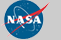Curator: David M. Giles NASA Official: Brent N. Holben Generated: 10/12/2019